# RD Sharma Solutions for Class 9 Maths Chapter 1 Number System

## RD Sharma Solutions for Class 9 Maths Chapter 1 – Free PDF Download

RD Sharma Solutions for Class 9 Maths Chapter 1 Number System are given here to help students secure high marks in exams. Chapter 1 of Class 9 Maths mainly deals with problems based on rational and irrational numbers, natural numbers, whole numbers, representation of real numbers and many more. In order to strengthen your basic fundamentals on the number system, students must practice RD Sharma Solutions on a daily basis. Students can download all chapters of RD Sharma Class 9 Solutions both online and offline mode.

RD Sharma textbook for Class 9 is based on the latest syllabus prescribed by the CBSE that is the Central Board of Secondary Education. In this textbook, at the end of each chapter, an exercise consisting of multiple-choice questions and summary for quick revision of concepts and formulae have been given. In order to excel in board exams, it is suggested to refer to RD Sharma Solutions for Class 9 while practising textbook problems.

## Download PDF of RD Sharma Solutions for Class 9 Maths Chapter 1 Number System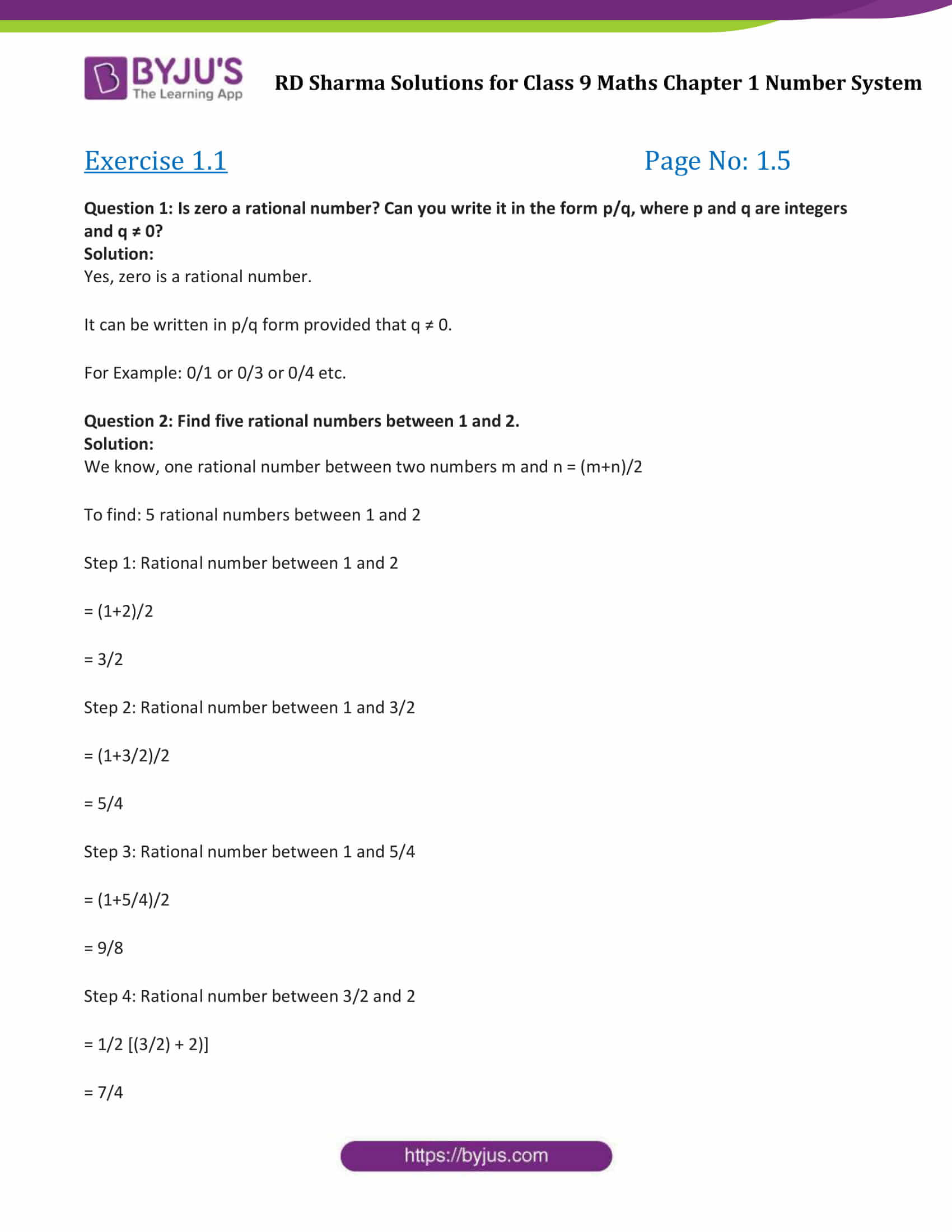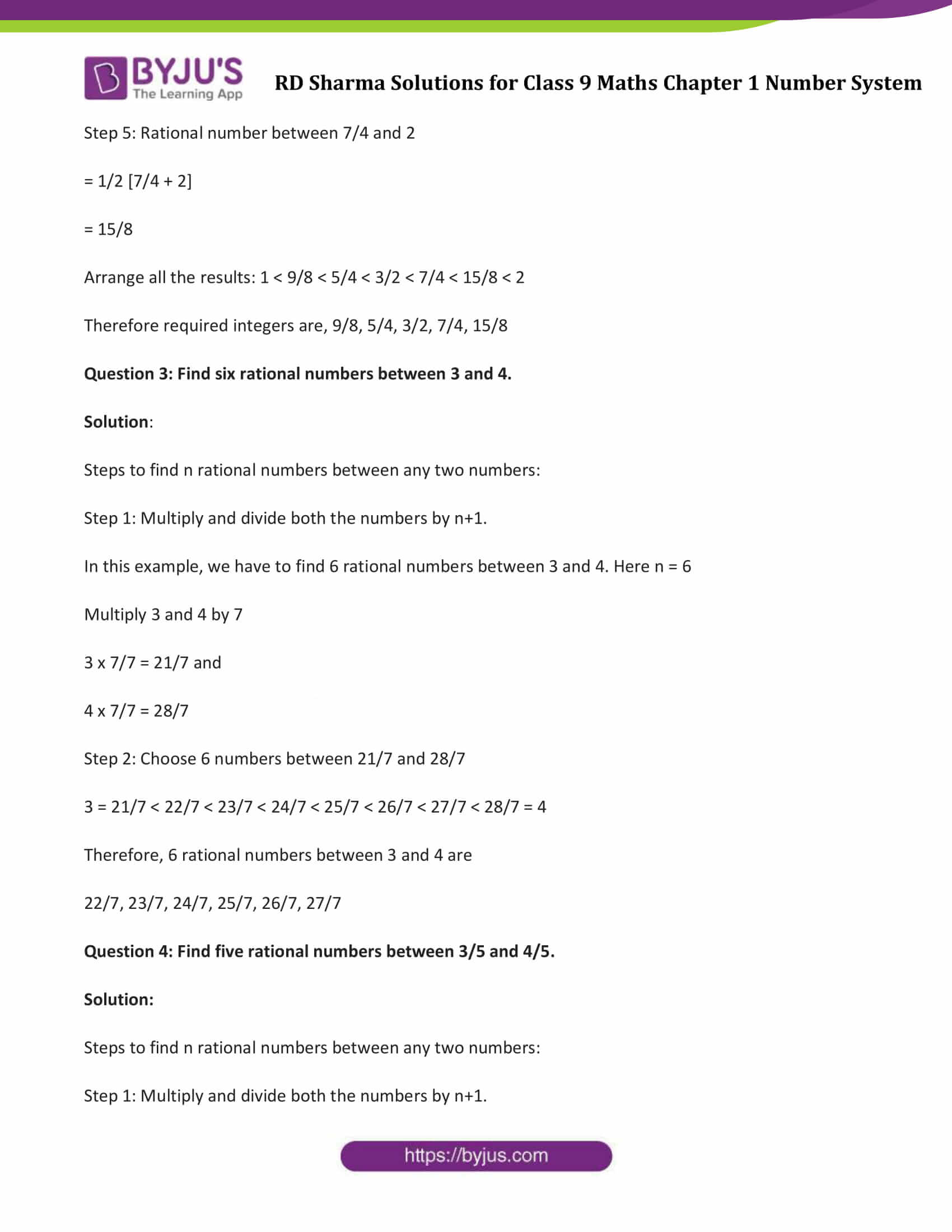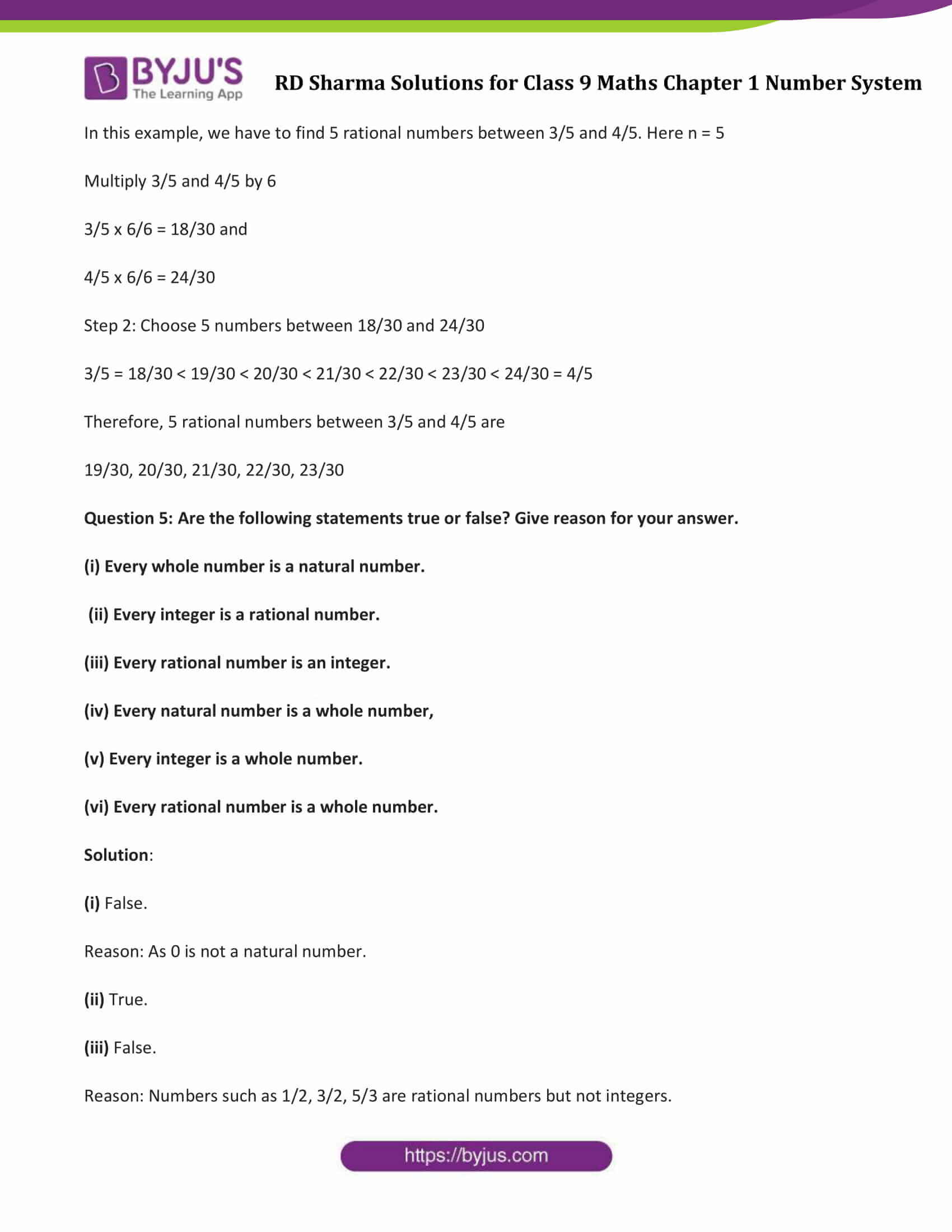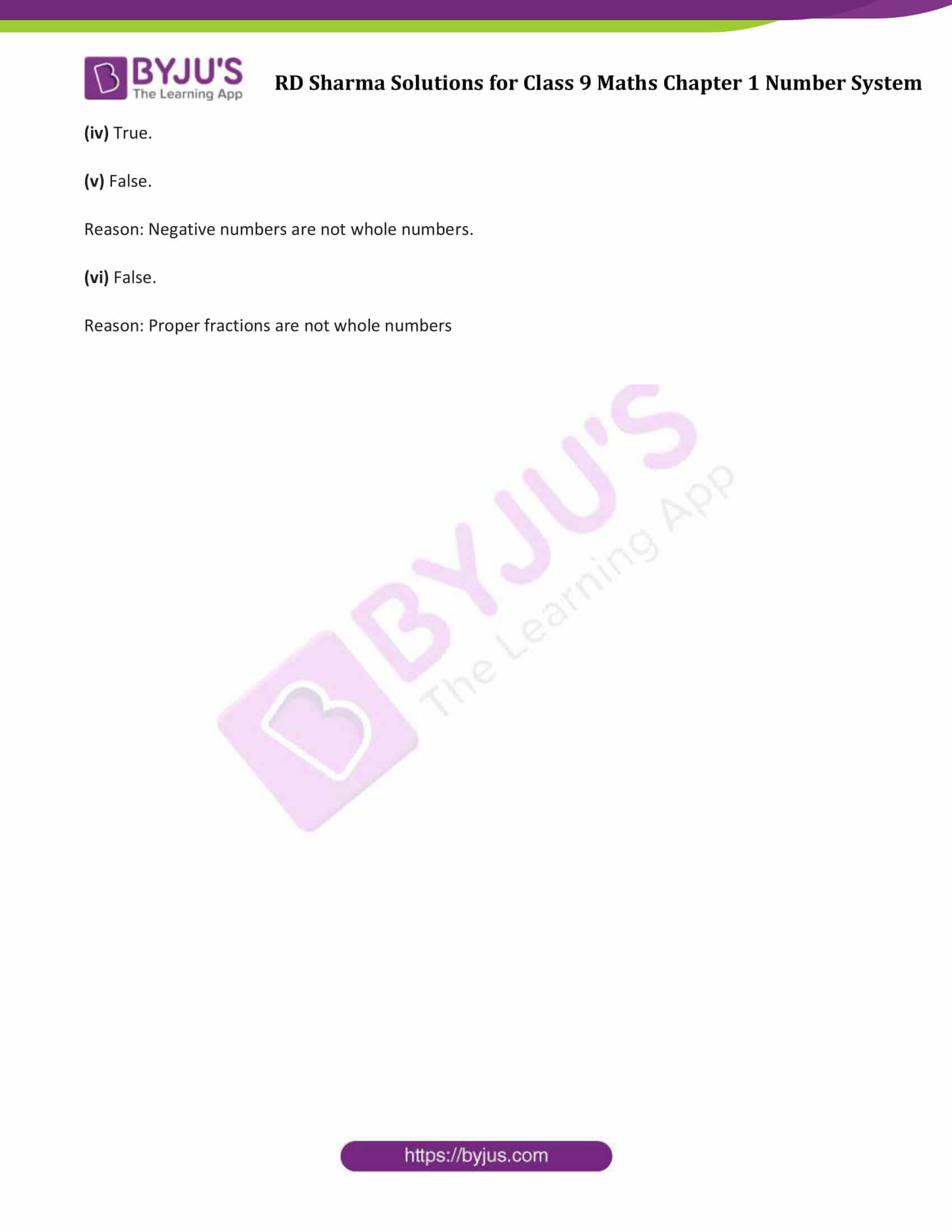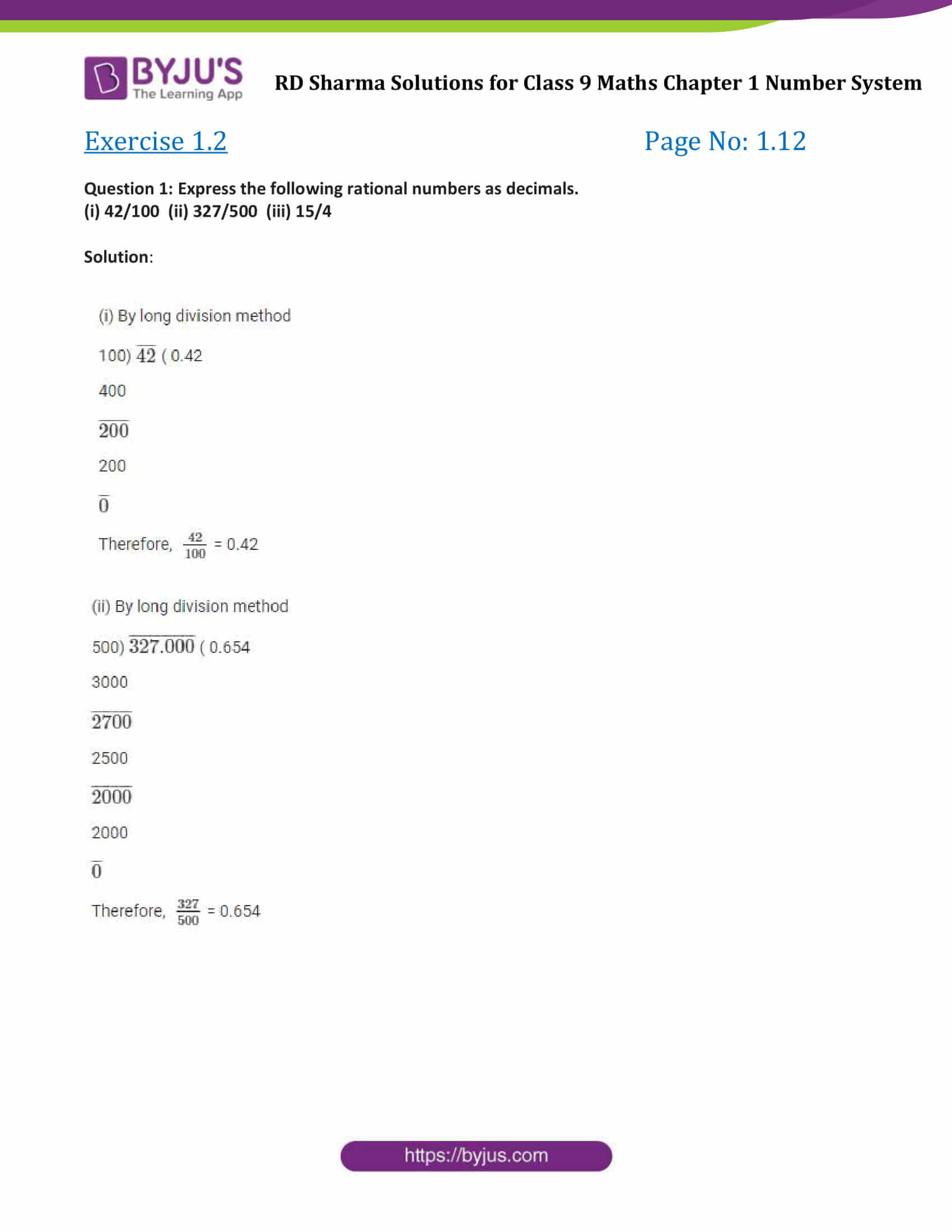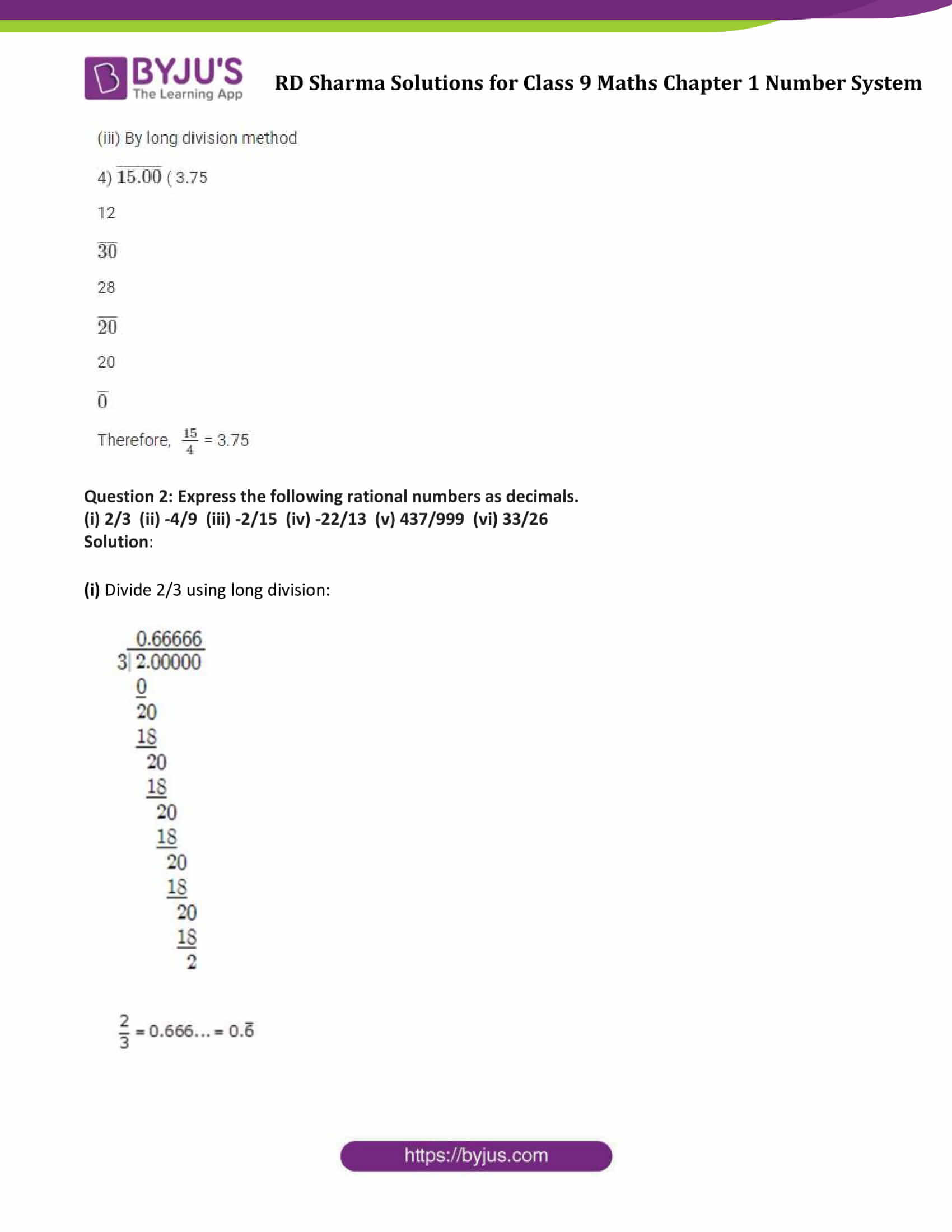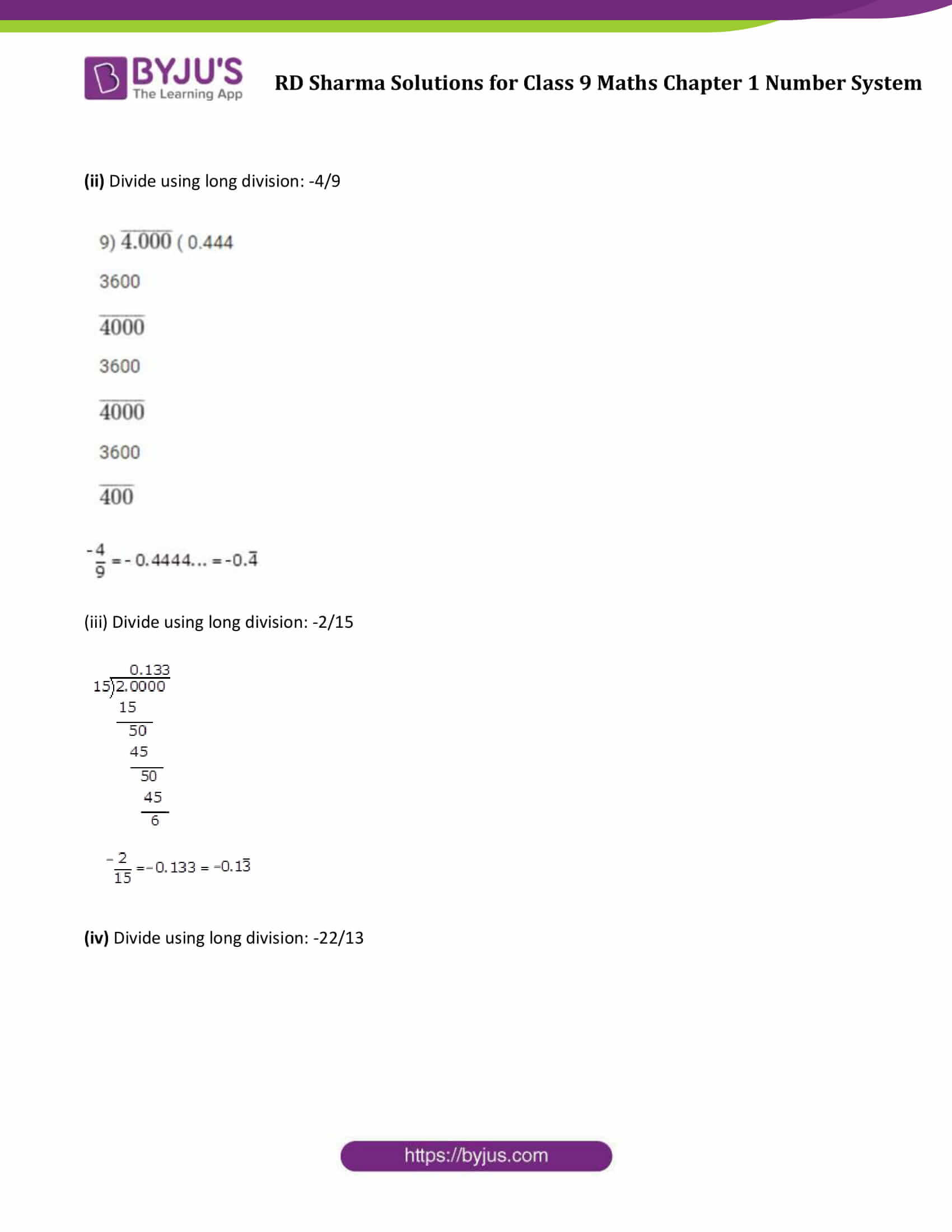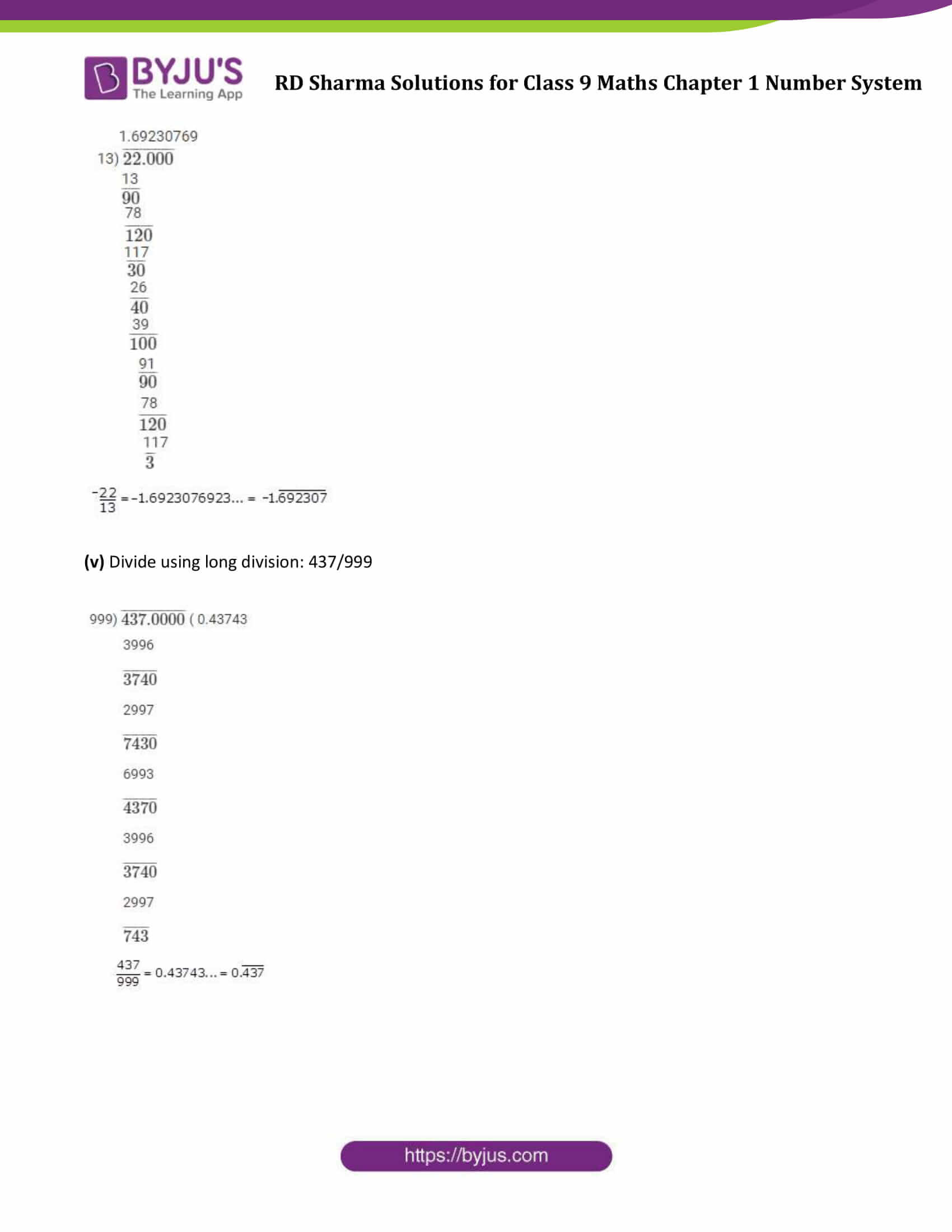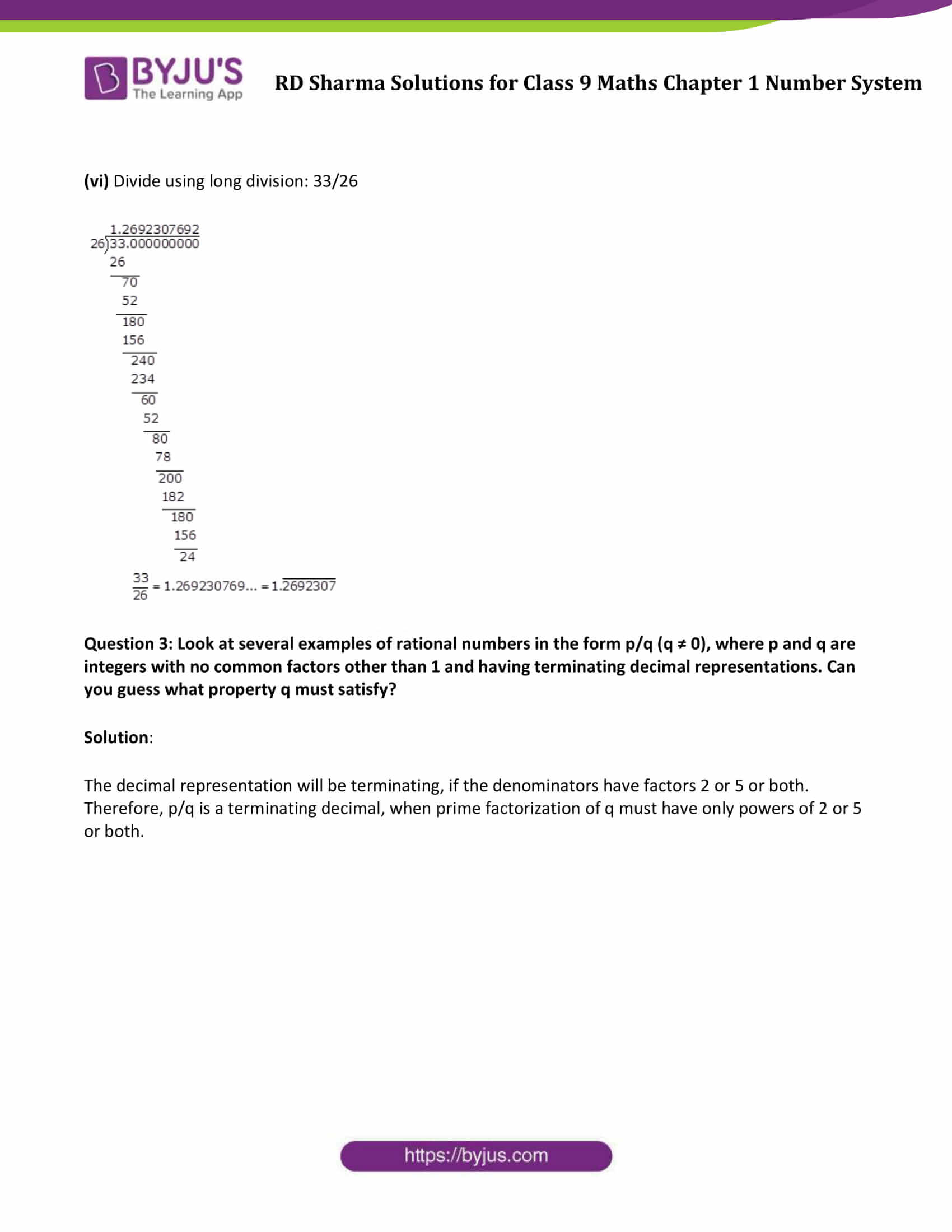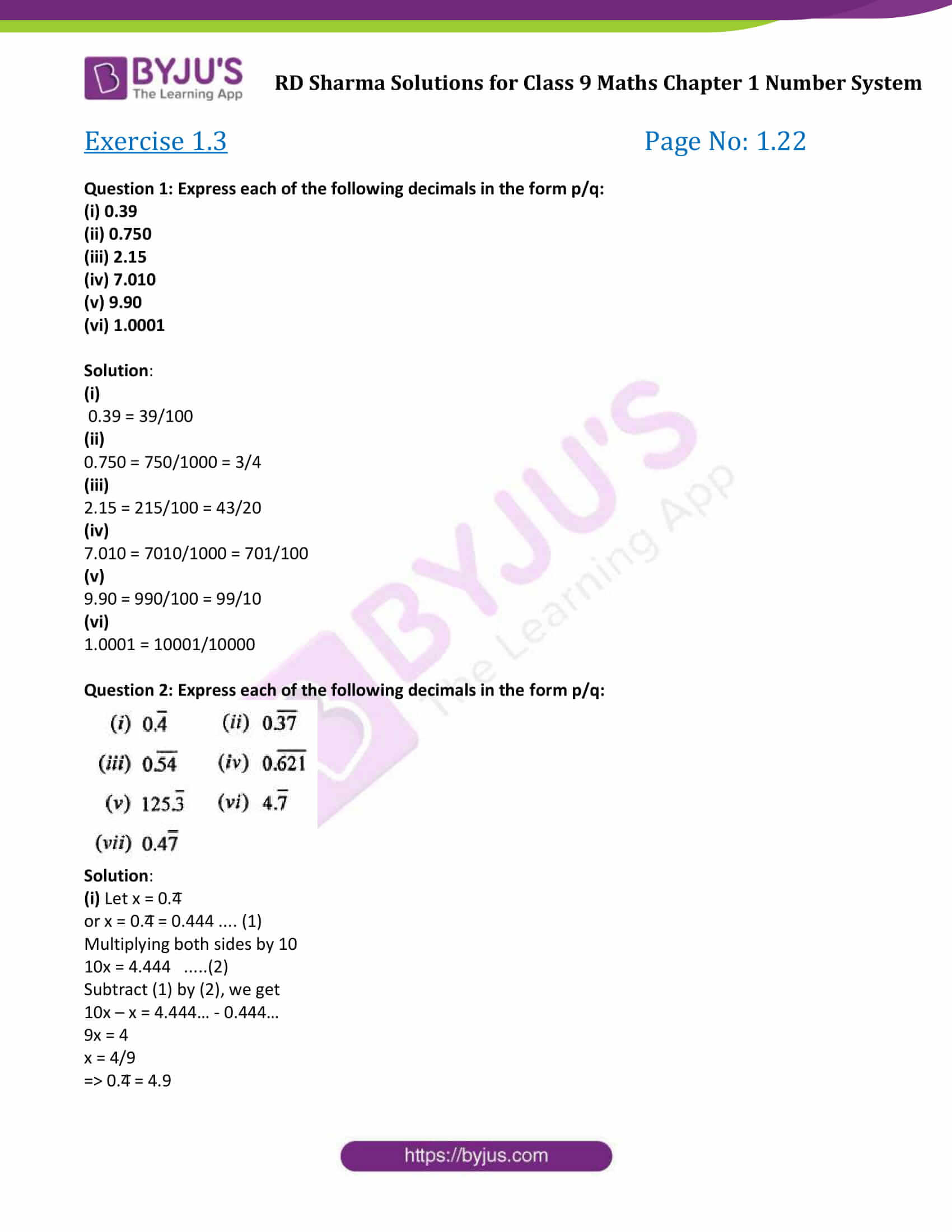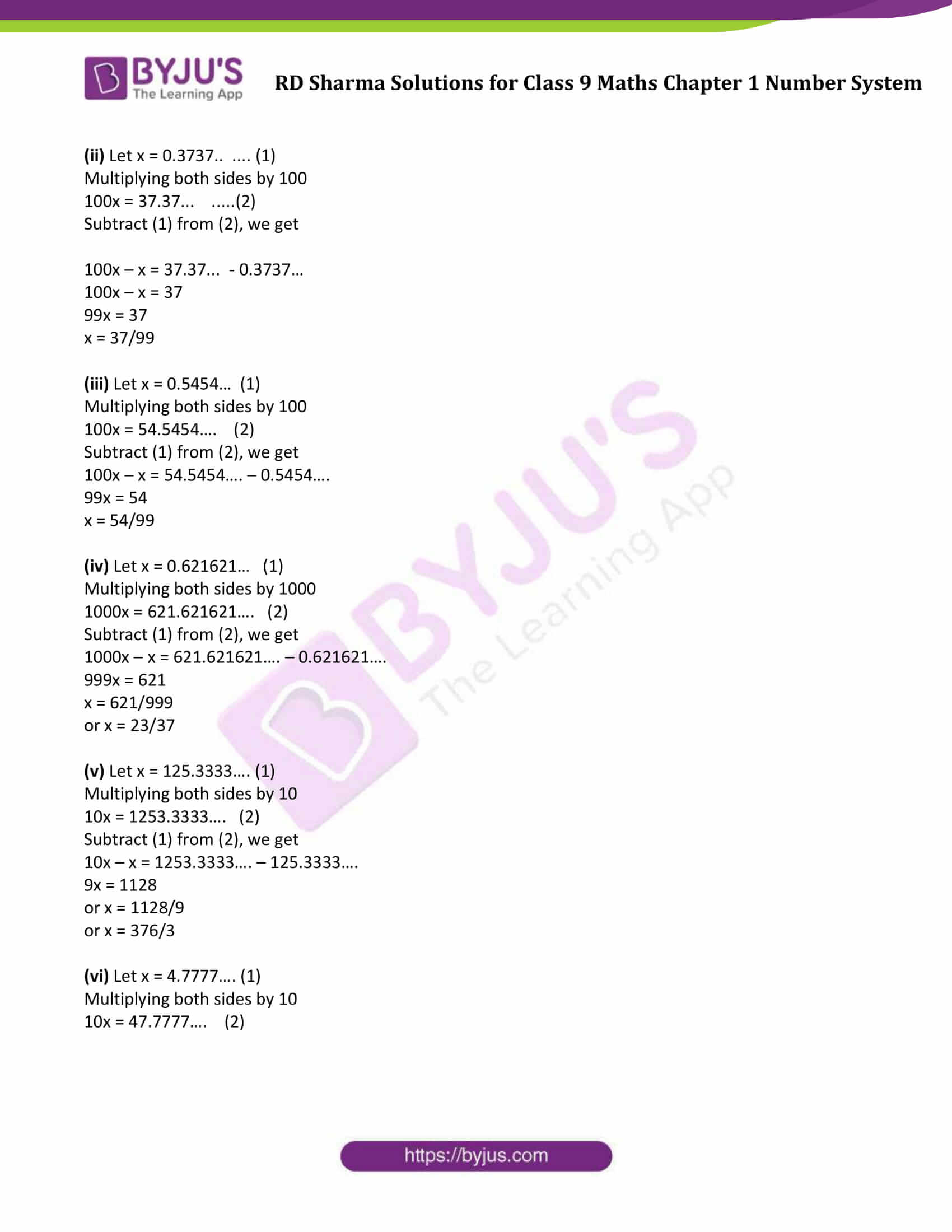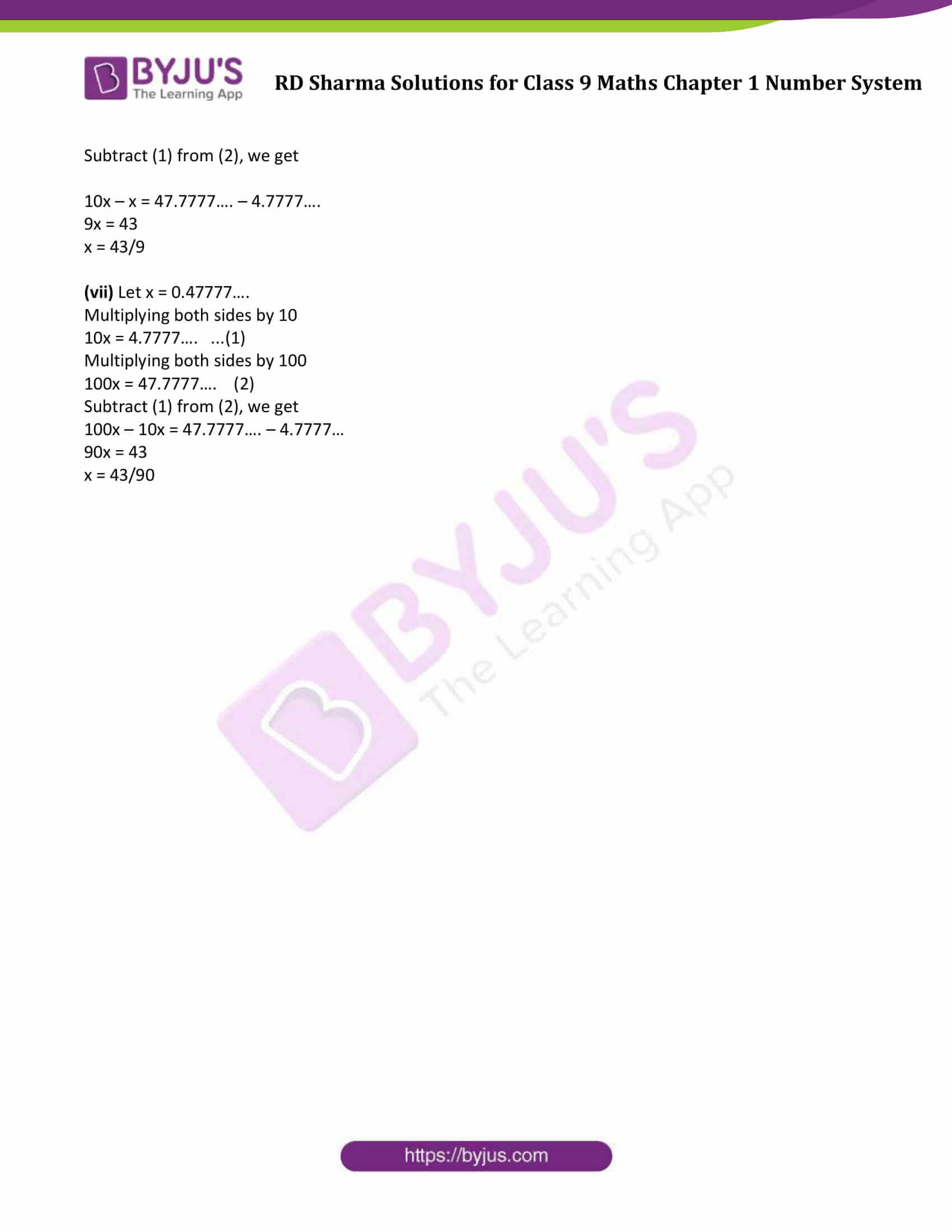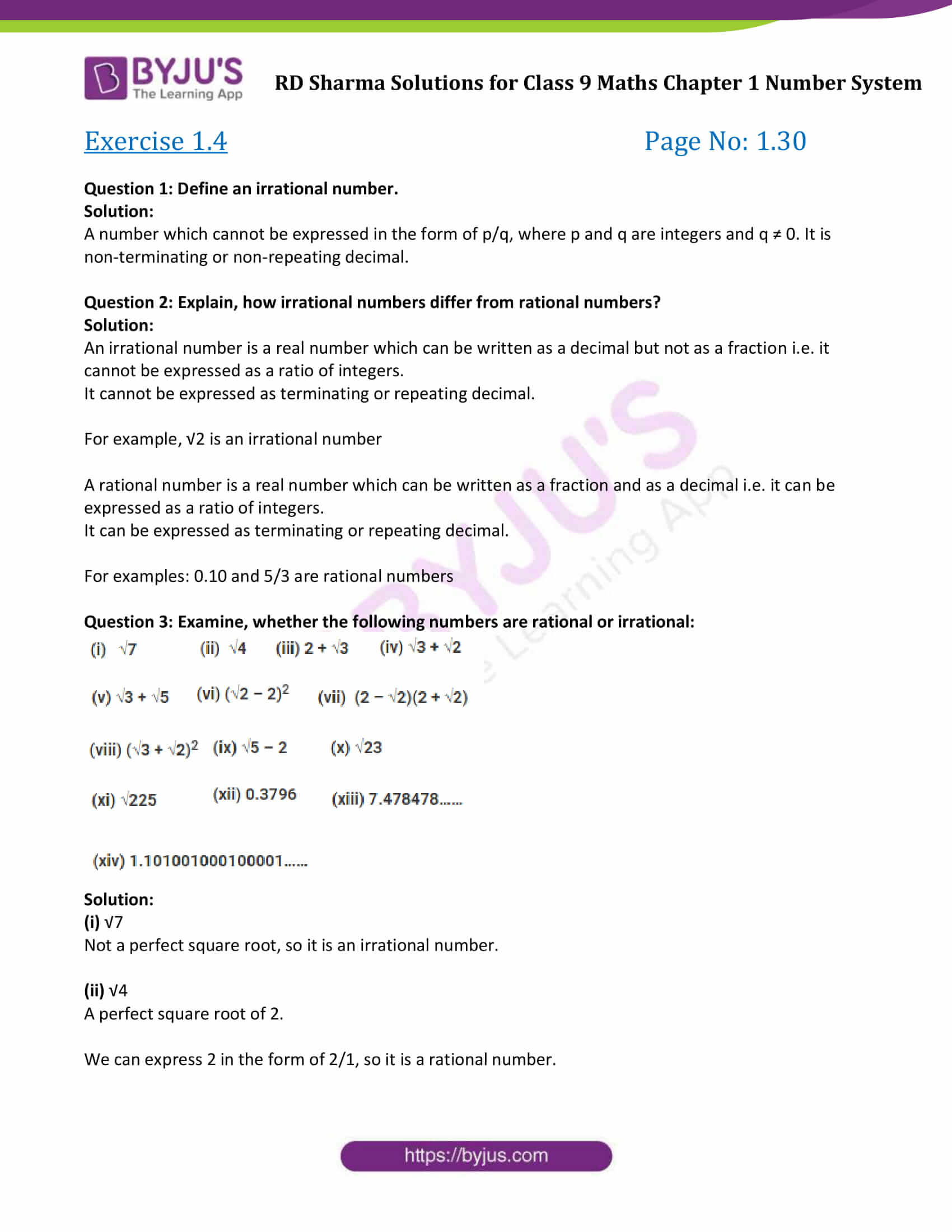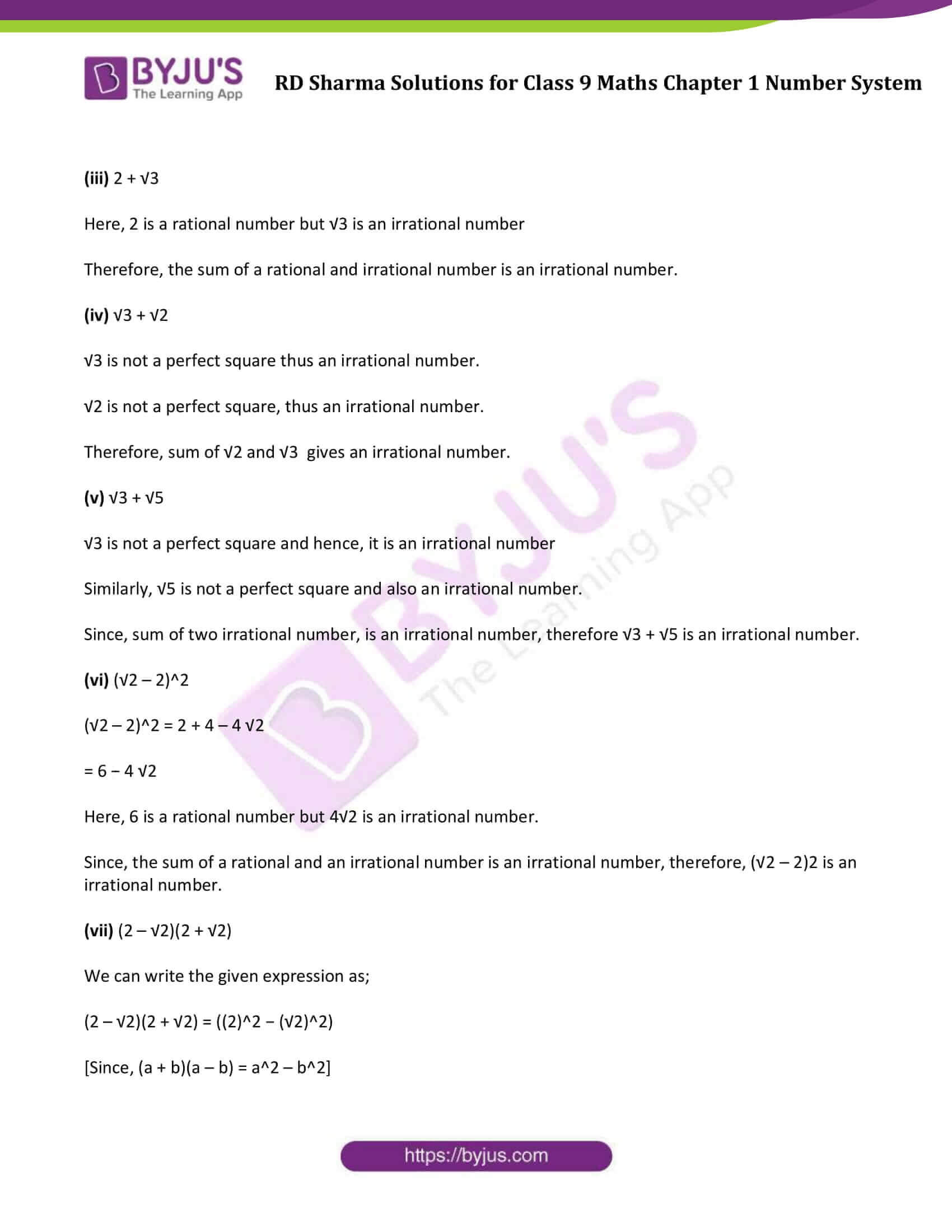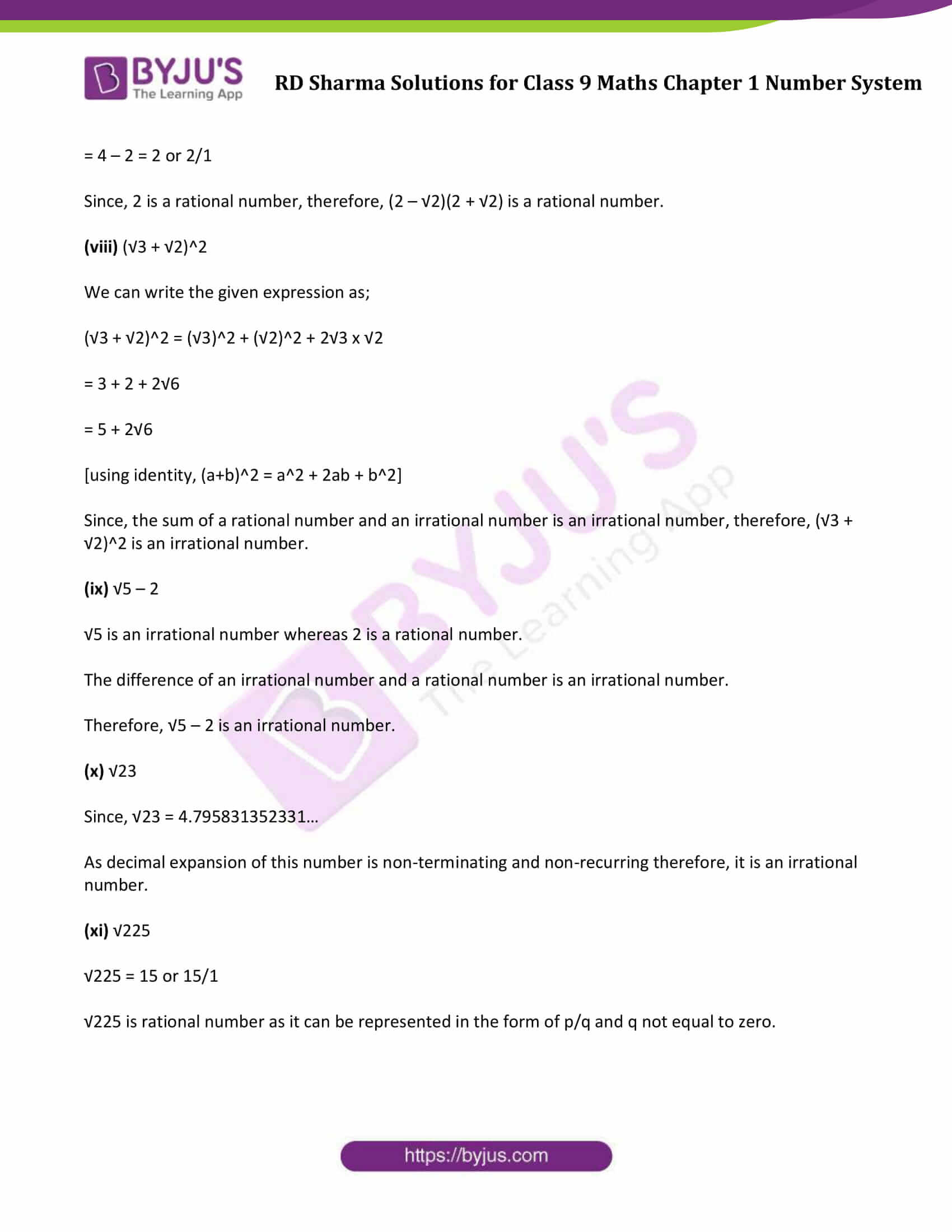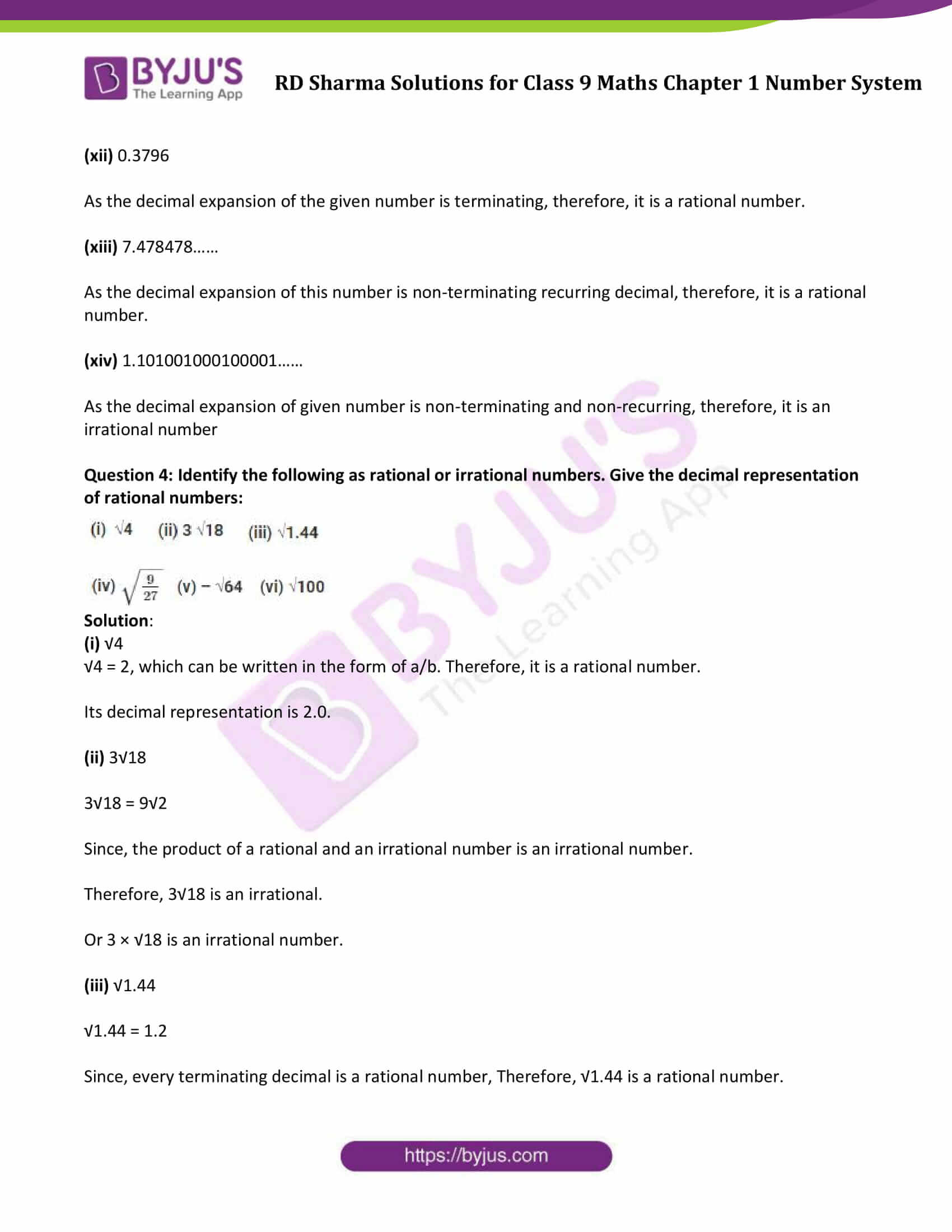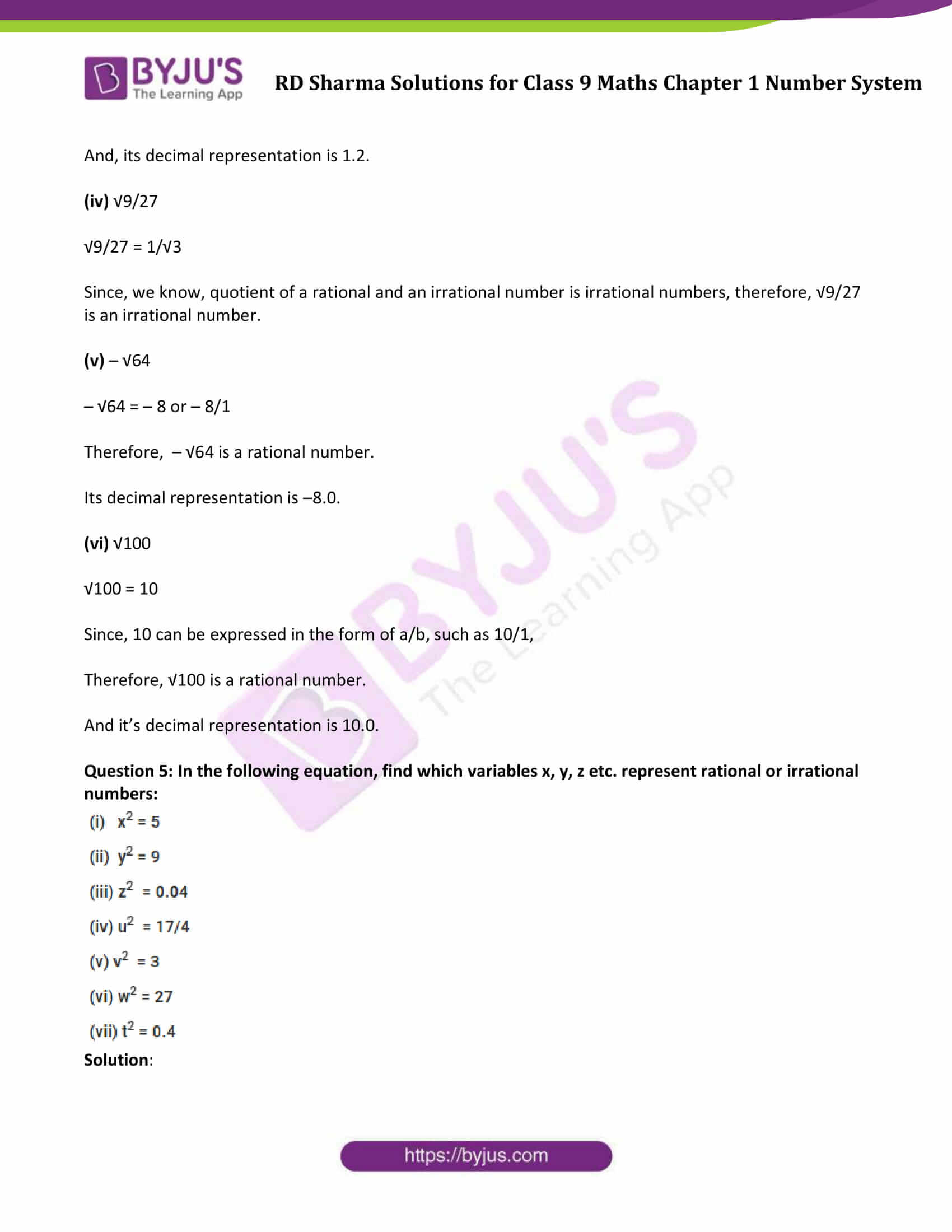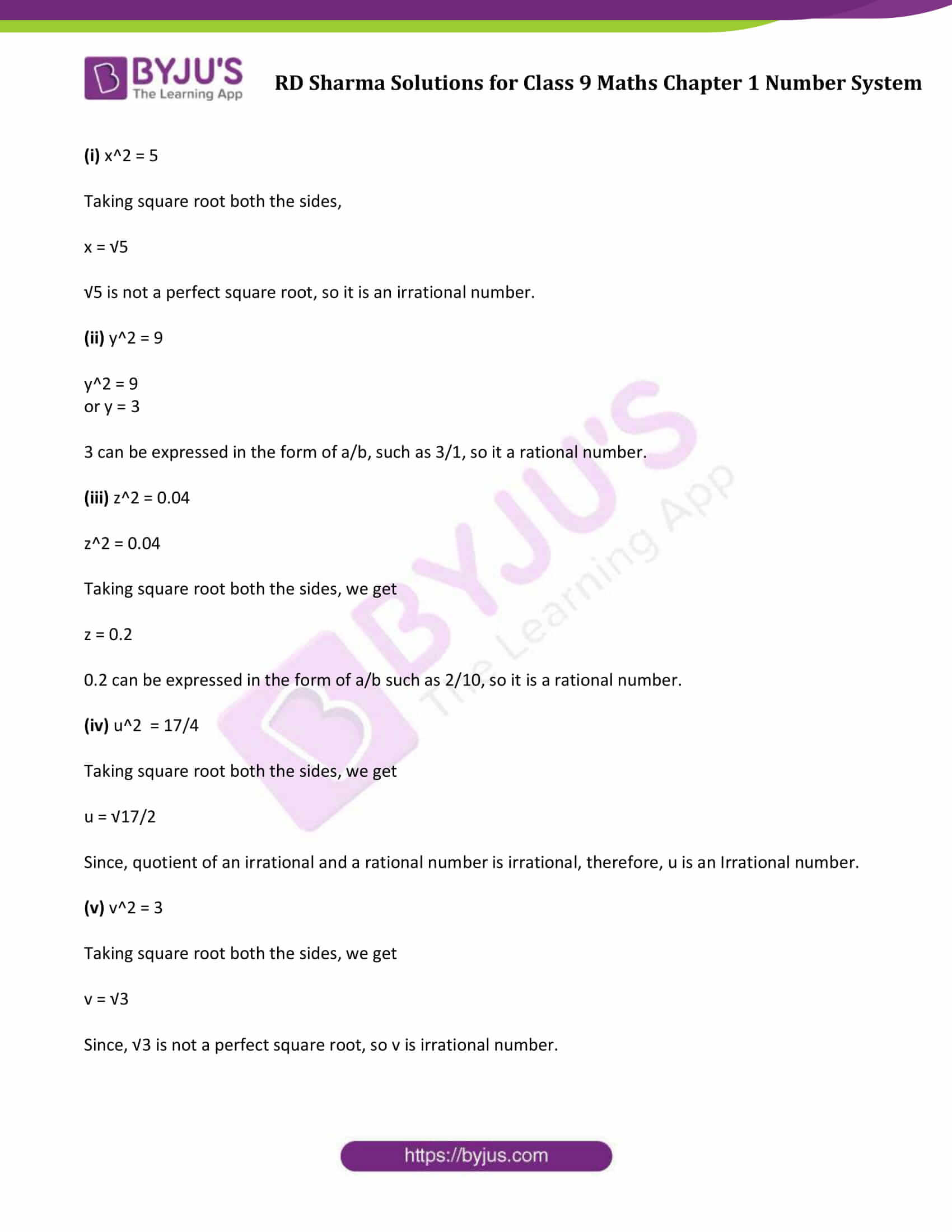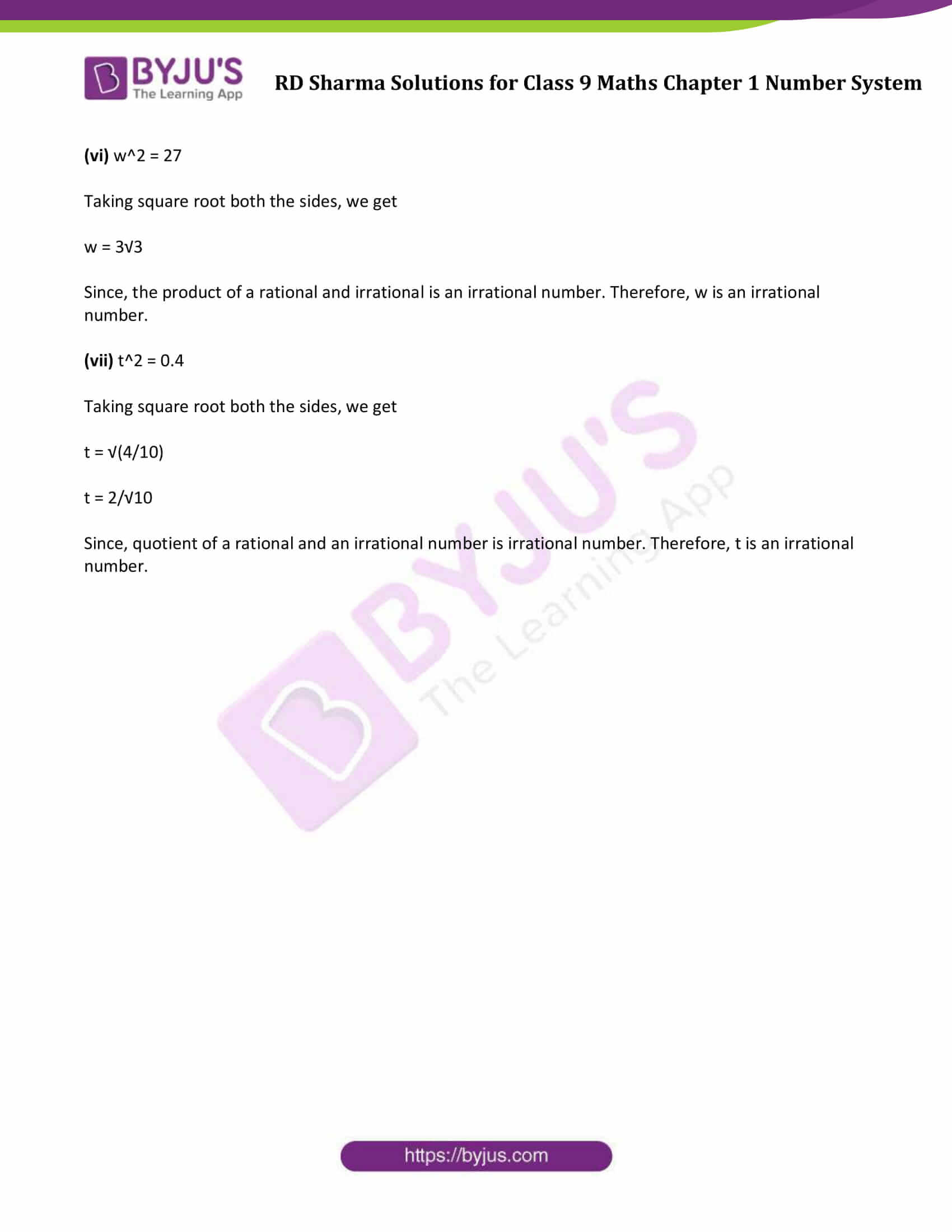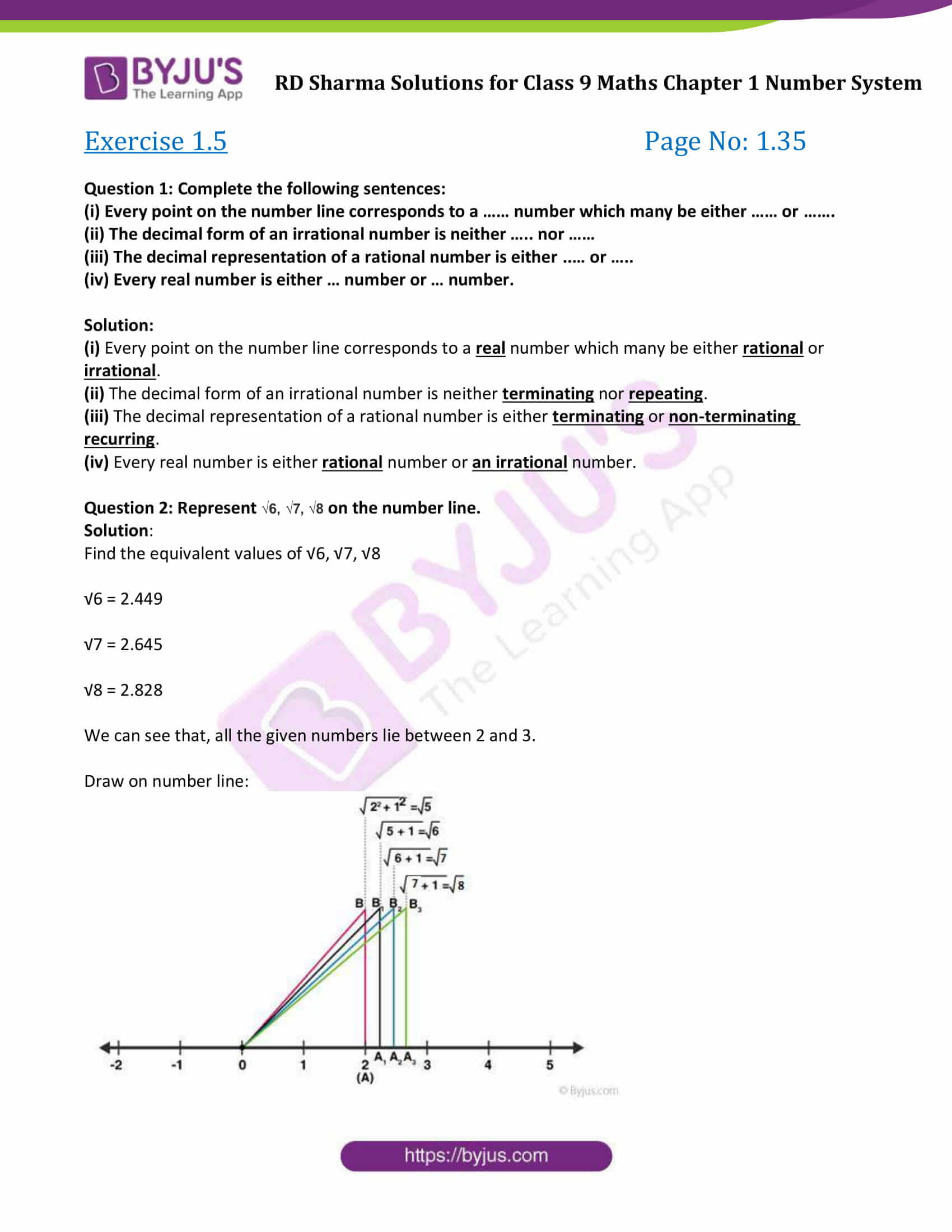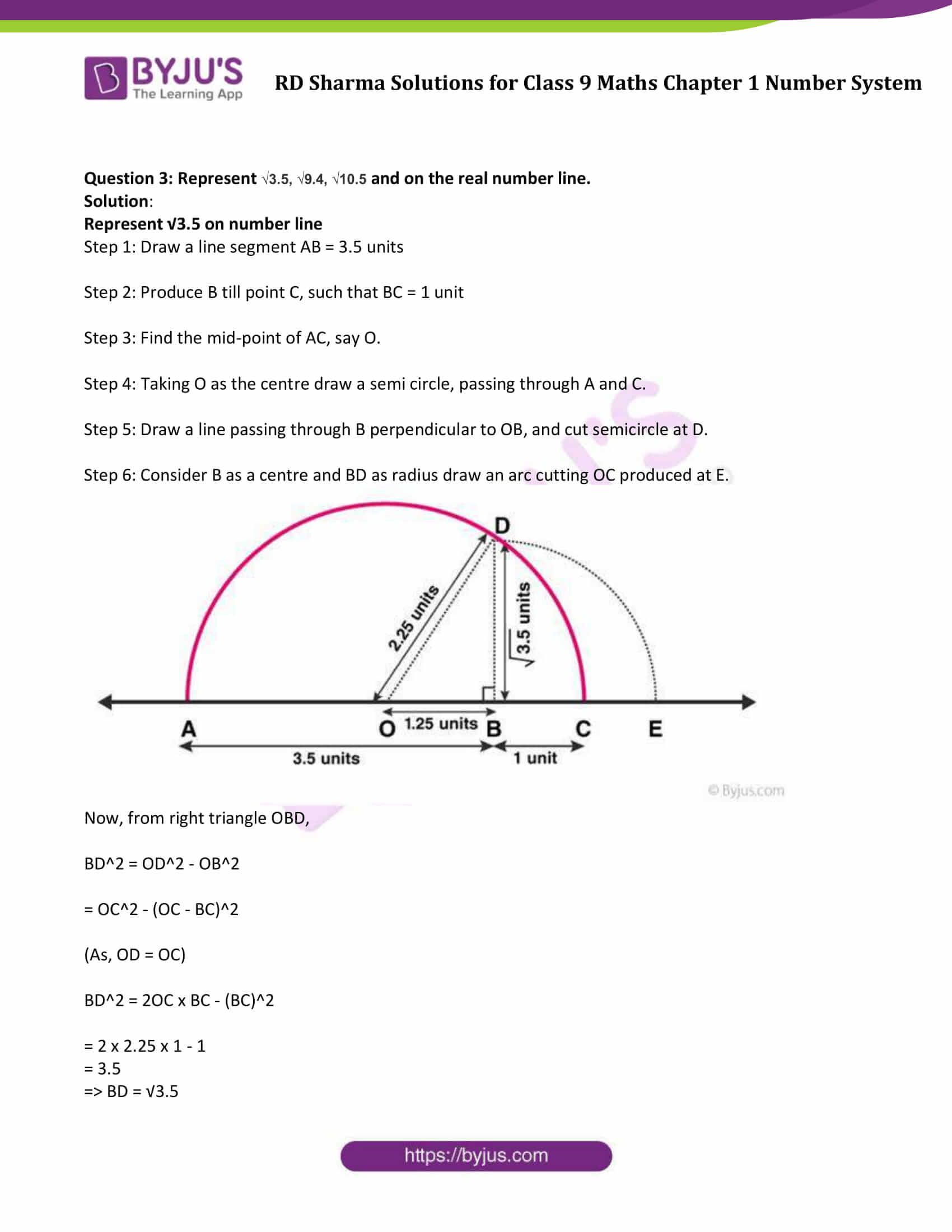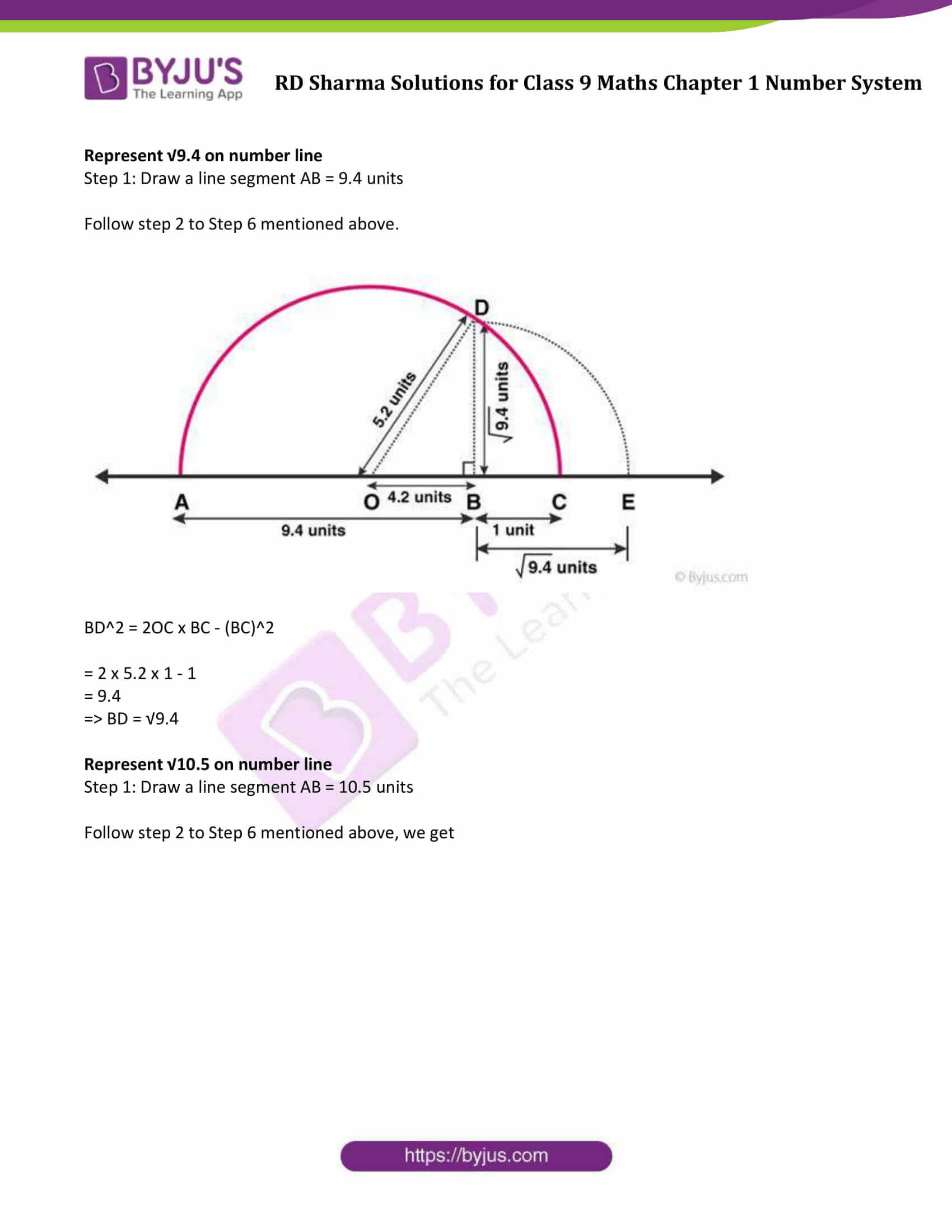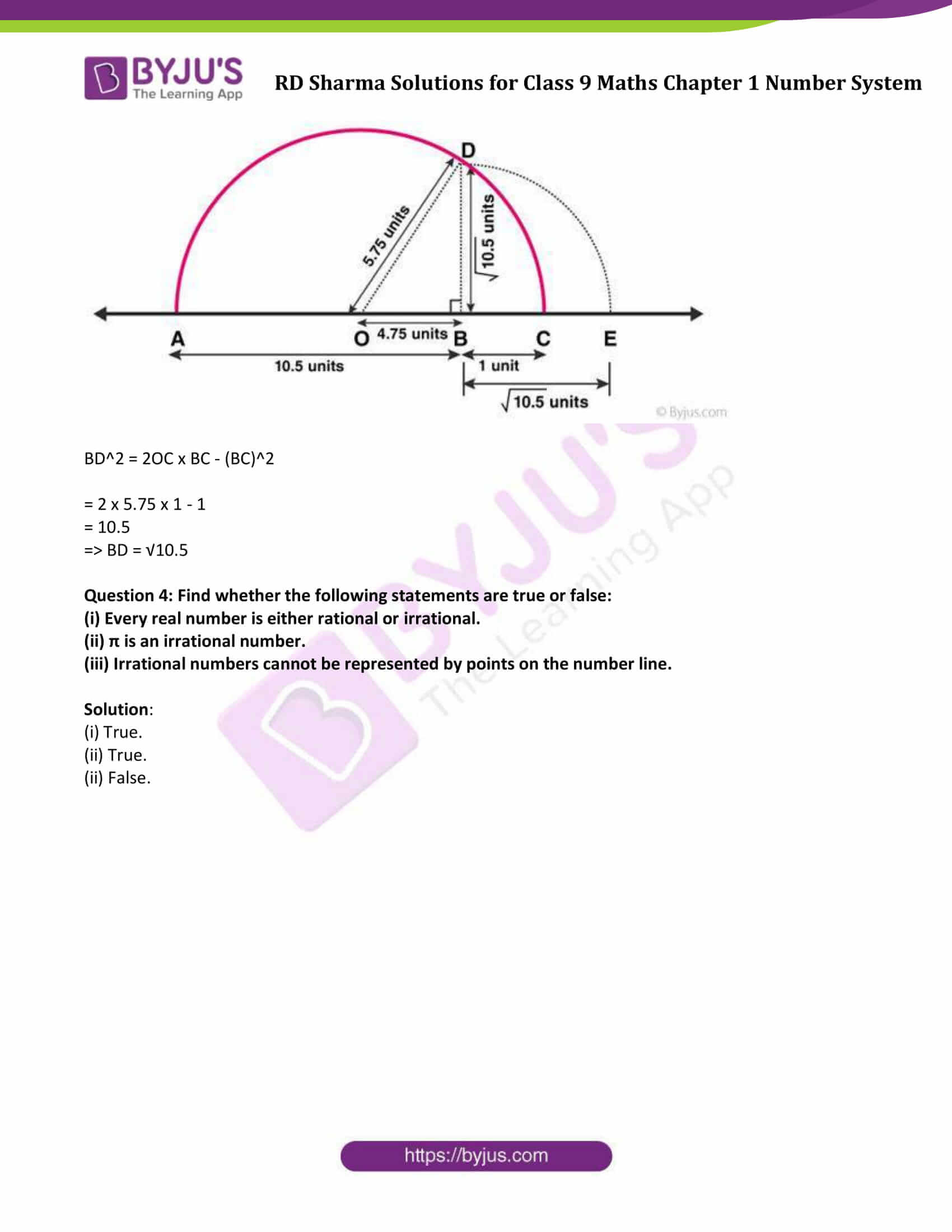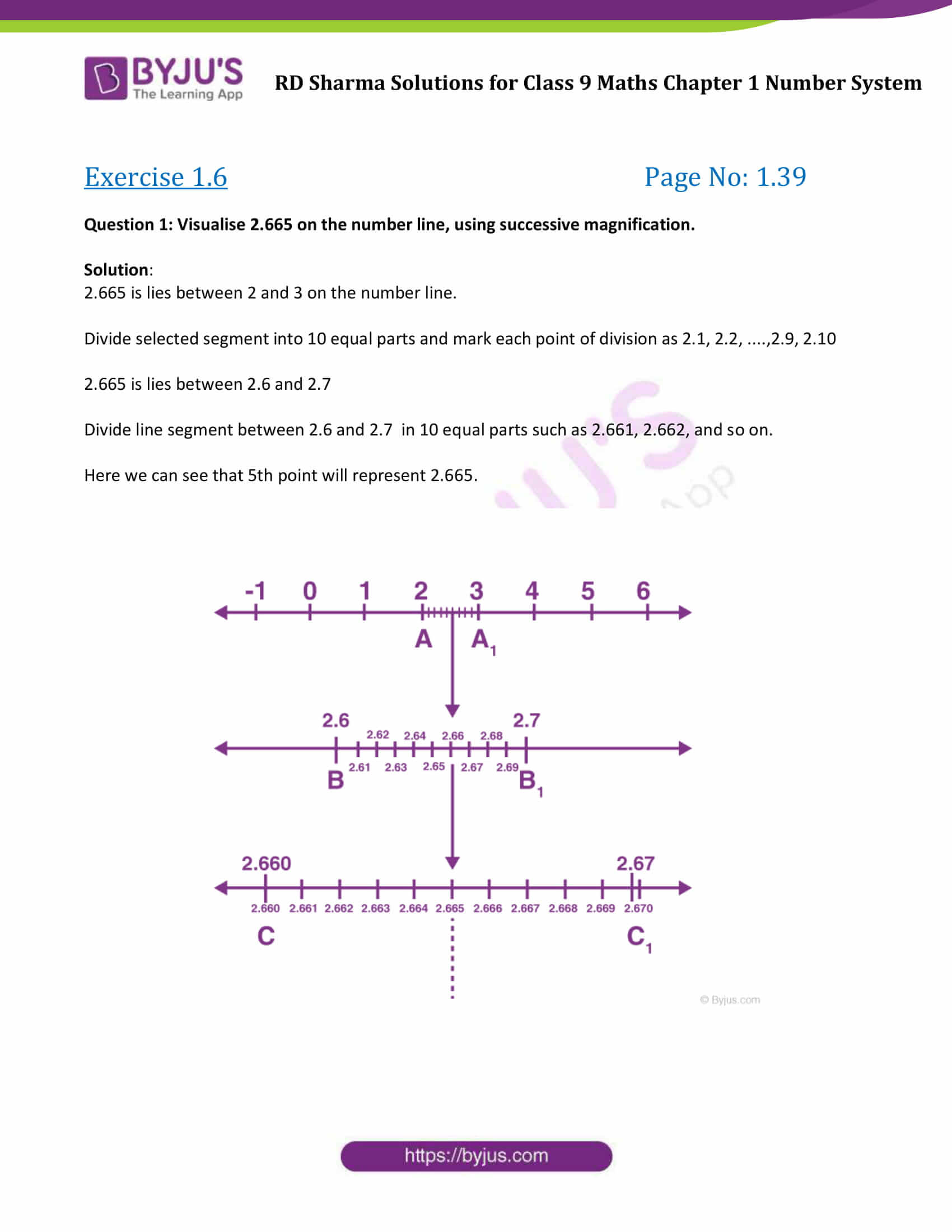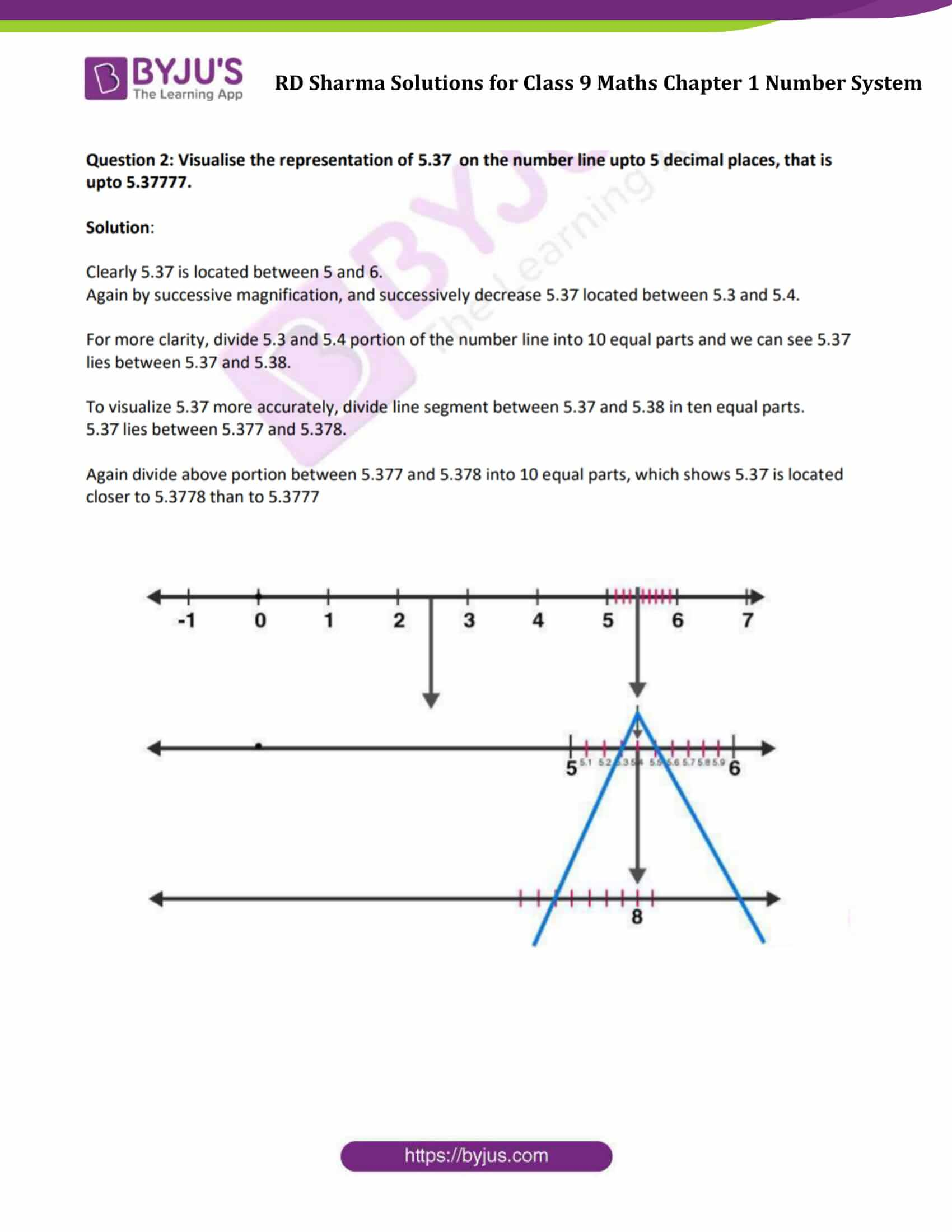### Exercise 1.1

Question 1: Is zero a rational number? Can you write it in the form p/q, where p and q are integers and q ≠ 0?
Solution:

Yes, zero is a rational number.

It can be written in p/q form provided that q ≠ 0.

For Example: 0/1 or 0/3 or 0/4 etc.

Question 2: Find five rational numbers between 1 and 2.
Solution:

We know, one rational number between two numbers m and n = (m+n)/2

To find: 5 rational numbers between 1 and 2

Step 1: Rational number between 1 and 2

= (1+2)/2

= 3/2

Step 2: Rational number between 1 and 3/2

= (1+3/2)/2

= 5/4

Step 3: Rational number between 1 and 5/4

= (1+5/4)/2

= 9/8

Step 4: Rational number between 3/2 and 2

= 1/2 [(3/2) + 2)]

= 7/4

Step 5: Rational number between 7/4 and 2

= 1/2 [7/4 + 2]

= 15/8

Arrange all the results: 1 < 9/8 < 5/4 < 3/2 < 7/4 < 15/8 < 2

Therefore required integers are, 9/8, 5/4, 3/2, 7/4, 15/8

Question 3: Find six rational numbers between 3 and 4.

Solution:

Steps to find n rational numbers between any two numbers:

Step 1: Multiply and divide both the numbers by n+1.

In this example, we have to find 6 rational numbers between 3 and 4. Here n = 6

Multiply 3 and 4 by 7

3 x 7/7 = 21/7 and

4 x 7/7 = 28/7

Step 2: Choose 6 numbers between 21/7 and 28/7

3 = 21/7 < 22/7 < 23/7 < 24/7 < 25/7 < 26/7 < 27/7 < 28/7 = 4

Therefore, 6 rational numbers between 3 and 4 are

22/7, 23/7, 24/7, 25/7, 26/7, 27/7

Question 4: Find five rational numbers between 3/5 and 4/5.

Solution:

Steps to find n rational numbers between any two numbers:

Step 1: Multiply and divide both the numbers by n+1.

In this example, we have to find 5 rational numbers between 3/5 and 4/5. Here n = 5

Multiply 3/5 and 4/5 by 6

3/5 x 6/6 = 18/30 and

4/5 x 6/6 = 24/30

Step 2: Choose 5 numbers between 18/30 and 24/30

3/5 = 18/30 < 19/30 < 20/30 < 21/30 < 22/30 < 23/30 < 24/30 = 4/5

Therefore, 5 rational numbers between 3/5 and 4/5 are

19/30, 20/30, 21/30, 22/30, 23/30

Question 5: Are the following statements true or false? Give reason for your answer.

(i) Every whole number is a natural number.

(ii) Every integer is a rational number.

(iii) Every rational number is an integer.

(iv) Every natural number is a whole number,

(v) Every integer is a whole number.

(vi) Every rational number is a whole number.

Solution:

(i) False.

Reason: As 0 is not a natural number.

(ii) True.

(iii) False.

Reason: Numbers such as 1/2, 3/2, 5/3 are rational numbers but not integers.

(iv) True.

(v) False.

Reason: Negative numbers are not whole numbers.

(vi) False.

Reason: Proper fractions are not whole numbers.

### Exercise 1.2

Question 1: Express the following rational numbers as decimals.
(i) 42/100 (ii) 327/500 (iii) 15/4

Solution: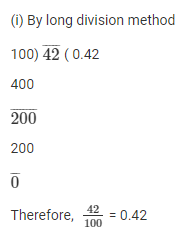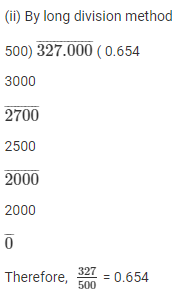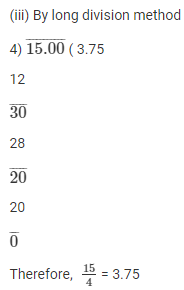Question 2: Express the following rational numbers as decimals.
(i) 2/3 (ii) -4/9 (iii) -2/15 (iv) -22/13 (v) 437/999 (vi) 33/26
Solution
:

(i) Divide 2/3 using long division: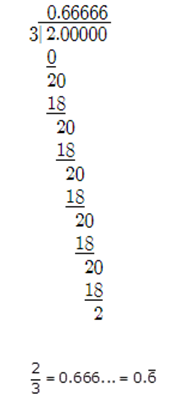(ii) Divide using long division: -4/9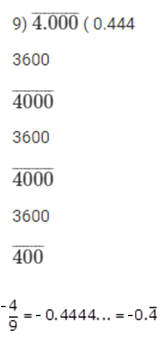(iii) Divide using long division: -2/15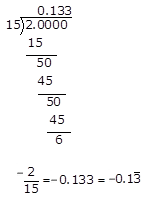(iv) Divide using long division: -22/13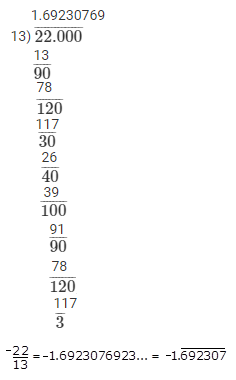(v) Divide using long division: 437/999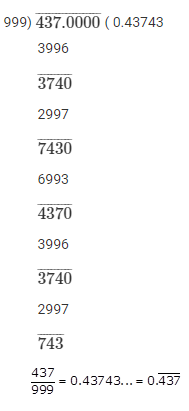(vi) Divide using long division: 33/26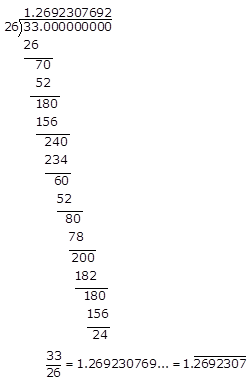Question 3: Look at several examples of rational numbers in the form p/q (q ≠ 0), where p and q are integers with no common factors other than 1 and having terminating decimal representations. Can you guess what property q must satisfy?

Solution:

The decimal representation will be terminating, if the denominators have factors 2 or 5 or both. Therefore, p/q is a terminating decimal, when prime factorization of q must have only powers of 2 or 5 or both.

### Exercise 1.3

Question 1: Express each of the following decimals in the form p/q:

(i) 0.39

(ii) 0.750

(iii) 2.15

(iv) 7.010

(v) 9.90

(vi) 1.0001

Solution:

(i)

0.39 = 39/100

(ii)

0.750 = 750/1000 = 3/4

(iii)

2.15 = 215/100 = 43/20

(iv)

7.010 = 7010/1000 = 701/100

(v)

9.90 = 990/100 = 99/10

(vi)

1.0001 = 10001/10000

Question 2: Express each of the following decimals in the form p/q: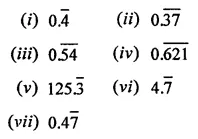Solution:

(i) Let x = 0.4̅

or x = 0.4̅ = 0.444 …. (1)

Multiplying both sides by 10

10x = 4.444 …..(2)

Subtract (1) by (2), we get

10x – x = 4.444… – 0.444…

9x = 4

x = 4/9

=> 0.4̅ = 4.9

(ii) Let x = 0.3737.. …. (1)

Multiplying both sides by 100

100x = 37.37… …..(2)

Subtract (1) from (2), we get

100x – x = 37.37… – 0.3737…

100x – x = 37

99x = 37

x = 37/99

(iii) Let x = 0.5454… (1)

Multiplying both sides by 100

100x = 54.5454…. (2)

Subtract (1) from (2), we get

100x – x = 54.5454…. – 0.5454….

99x = 54

x = 54/99

(iv) Let x = 0.621621… (1)

Multiplying both sides by 1000

1000x = 621.621621…. (2)

Subtract (1) from (2), we get

1000x – x = 621.621621…. – 0.621621….

999x = 621

x = 621/999

or x = 23/37

(v) Let x = 125.3333…. (1)

Multiplying both sides by 10

10x = 1253.3333…. (2)

Subtract (1) from (2), we get

10x – x = 1253.3333…. – 125.3333….

9x = 1128

or x = 1128/9

or x = 376/3

(vi) Let x = 4.7777…. (1)

Multiplying both sides by 10

10x = 47.7777…. (2)

Subtract (1) from (2), we get

10x – x = 47.7777…. – 4.7777….

9x = 43

x = 43/9

(vii) Let x = 0.47777….

Multiplying both sides by 10

10x = 4.7777…. …(1)

Multiplying both sides by 100

100x = 47.7777…. (2)

Subtract (1) from (2), we get

100x – 10x = 47.7777…. – 4.7777…

90x = 43

x = 43/90

### Exercise 1.4

Question 1: Define an irrational number.

Solution:

A number which cannot be expressed in the form of p/q, where p and q are integers and q ≠ 0. It is non-terminating or non-repeating decimal.

Question 2: Explain, how irrational numbers differ from rational numbers?

Solution:

An irrational number is a real number which can be written as a decimal but not as a fraction i.e. it cannot be expressed as a ratio of integers.

It cannot be expressed as terminating or repeating decimal.

For example, √2 is an irrational number

A rational number is a real number which can be written as a fraction and as a decimal i.e. it can be expressed as a ratio of integers.

It can be expressed as terminating or repeating decimal.

For examples: 0.10 and 5/3 are rational numbers

Question 3: Examine, whether the following numbers are rational or irrational: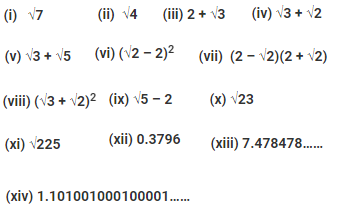Solution:

(i) √7

Not a perfect square root, so it is an irrational number.

(ii) √4

A perfect square root of 2.

We can express 2 in the form of 2/1, so it is a rational number.

(iii) 2 + √3

Here, 2 is a rational number but √3 is an irrational number

Therefore, the sum of a rational and irrational number is an irrational number.

(iv) √3 + √2

√3 is not a perfect square thus an irrational number.

√2 is not a perfect square, thus an irrational number.

Therefore, sum of √2 and √3 gives an irrational number.

(v) √3 + √5

√3 is not a perfect square and hence, it is an irrational number

Similarly, √5 is not a perfect square and also an irrational number.

Since, sum of two irrational number, is an irrational number, therefore √3 + √5 is an irrational number.

(vi) (√2 – 2)2

(√2 – 2)2 = 2 + 4 – 4 √2

= 6 – 4 √2

Here, 6 is a rational number but 4√2 is an irrational number.

Since, the sum of a rational and an irrational number is an irrational number, therefore, (√2 – 2)2 is an irrational number.

(vii) (2 – √2)(2 + √2)

We can write the given expression as;

(2 – √2)(2 + √2) = ((2)2 − (√2)2)

[Since, (a + b)(a – b) = a2 – b2]

= 4 – 2 = 2 or 2/1

Since, 2 is a rational number, therefore, (2 – √2)(2 + √2) is a rational number.

(viii) (√3 + √2)2

We can write the given expression as;

(√3 + √2)2 = (√3)2 + (√2)2 + 2√3 x √2

= 3 + 2 + 2√6

= 5 + 2√6

[using identity, (a+b)2 = a2 + 2ab + b2]

Since, the sum of a rational number and an irrational number is an irrational number, therefore, (√3 + √2)2 is an irrational number.

(ix) √5 – 2

√5 is an irrational number whereas 2 is a rational number.

The difference of an irrational number and a rational number is an irrational number.

Therefore, √5 – 2 is an irrational number.

(x) √23

Since, √23 = 4.795831352331…

As decimal expansion of this number is non-terminating and non-recurring therefore, it is an irrational number.

(xi) √225

√225 = 15 or 15/1

√225 is rational number as it can be represented in the form of p/q and q not equal to zero.

(xii) 0.3796

As the decimal expansion of the given number is terminating, therefore, it is a rational number.

(xiii) 7.478478……

As the decimal expansion of this number is non-terminating recurring decimal, therefore, it is a rational number.

(xiv) 1.101001000100001……

As the decimal expansion of given number is non-terminating and non-recurring, therefore, it is an irrational number.

Question 4: Identify the following as rational or irrational numbers. Give the decimal representation of rational numbers: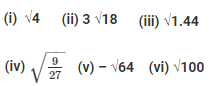Solution:

(i) √4

√4 = 2, which can be written in the form of a/b. Therefore, it is a rational number.

Its decimal representation is 2.0.

(ii) 3√18

3√18 = 9√2

Since, the product of a rational and an irrational number is an irrational number.

Therefore, 3√18 is an irrational.

Or 3 × √18 is an irrational number.

(iii) √1.44

√1.44 = 1.2

Since, every terminating decimal is a rational number, Therefore, √1.44 is a rational number.

And, its decimal representation is 1.2.

(iv) √9/27

√9/27 = 1/√3

Since, we know, quotient of a rational and an irrational number is irrational numbers, therefore, √9/27 is an irrational number.

(v) – √64

– √64 = – 8 or – 8/1

Therefore, – √64 is a rational number.

Its decimal representation is –8.0.

(vi) √100

√100 = 10

Since, 10 can be expressed in the form of a/b, such as 10/1,

Therefore, √100 is a rational number.

And it’s decimal representation is 10.0.

Question 5: In the following equation, find which variables x, y, z etc. represent rational or irrational numbers: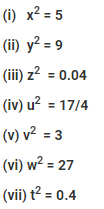Solution:

(i) x2 = 5

Taking square root both the sides,

x = √5

√5 is not a perfect square root, so it is an irrational number.

(ii) y2 = 9

y2 = 9

or y = 3

3 can be expressed in the form of a/b, such as 3/1, so it a rational number.

(iii) z2 = 0.04

z2 = 0.04

Taking square root both the sides, we get

z = 0.2

0.2 can be expressed in the form of a/b such as 2/10, so it is a rational number.

(iv) u2 = 17/4

Taking square root both the sides, we get

u = √17/2

Since, quotient of an irrational and a rational number is irrational, therefore, u is an Irrational number.

(v) v2 = 3

Taking square root both the sides, we get

v = √3

Since, √3 is not a perfect square root, so v is irrational number.

(vi) w2 = 27

Taking square root both the sides, we get

w = 3√3

Since, the product of a rational and irrational is an irrational number. Therefore, w is an irrational number.

(vii) t2 = 0.4

Taking square root both the sides, we get

t = √(4/10)

t = 2/√10

Since, quotient of a rational and an irrational number is irrational number. Therefore, t is an irrational number.

### Exercise 1.5

Question 1: Complete the following sentences:

(i) Every point on the number line corresponds to a …… number which many be either …… or …….

(ii) The decimal form of an irrational number is neither ….. nor ……

(iii) The decimal representation of a rational number is either ..… or …..

(iv) Every real number is either … number or … number.

Solution:

(i) Every point on the number line corresponds to a real number which many be either rational or irrational.

(ii) The decimal form of an irrational number is neither terminating nor repeating.

(iii) The decimal representation of a rational number is either terminating or non-terminating recurring.

(iv) Every real number is either rational number or an irrational number.

Question 2: Represent √6, √7, √8 on the number line.

Solution:

Find the equivalent values of √6, √7, √8

√6 = 2.449

√7 = 2.645

√8 = 2.828

We can see that, all the given numbers lie between 2 and 3.

Draw on number line: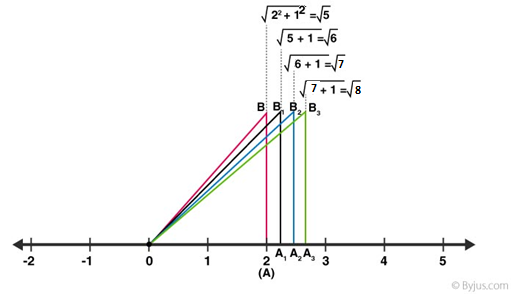Question 3: Represent √3.5, √9.4, √10.5 and on the real number line.

Solution:

Represent √3.5 on number line

Step 1: Draw a line segment AB = 3.5 units

Step 2: Produce B till point C, such that BC = 1 unit

Step 3: Find the mid-point of AC, say O.

Step 4: Taking O as the centre draw a semi circle, passing through A and C.

Step 5: Draw a line passing through B perpendicular to OB, and cut semicircle at D.

Step 6: Consider B as a centre and BD as radius draw an arc cutting OC produced at E.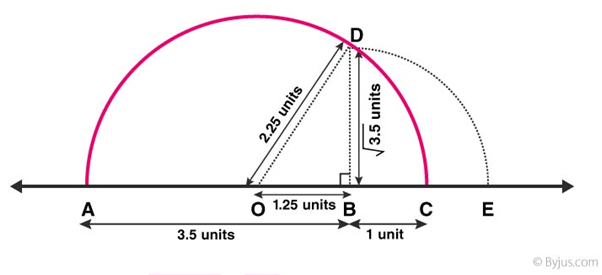Now, from right triangle OBD,

BD2 = OD2 – OB2

= OC2 – (OC – BC)2

(As, OD = OC)

BD2 = 2OC x BC – (BC)2

= 2 x 2.25 x 1 – 1

= 3.5

=> BD = √3.5

Represent √9.4 on number line

Step 1: Draw a line segment AB = 9.4 units

Follow step 2 to Step 6 mentioned above.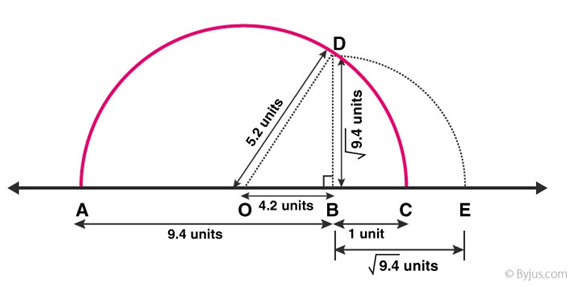BD2 = 2OC x BC – (BC)2

= 2 x 5.2 x 1 – 1

= 9.4

=> BD = √9.4

Represent √10.5 on number line

Step 1: Draw a line segment AB = 10.5 units

Follow step 2 to Step 6 mentioned above, we get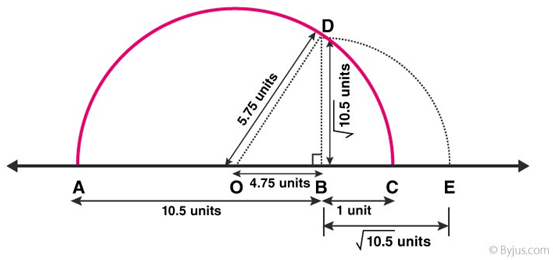BD2 = 2OC x BC – (BC)2

= 2 x 5.75 x 1 – 1

= 10.5

=> BD = √10.5

Question 4: Find whether the following statements are true or false:

(i) Every real number is either rational or irrational.

(ii) π is an irrational number.

(iii) Irrational numbers cannot be represented by points on the number line.

Solution:

(i) True.

(ii) True.

(ii) False.

### Exercise 1.6

Question 1: Visualise 2.665 on the number line, using successive magnification.

Solution:

2.665 is lies between 2 and 3 on the number line.

Divide selected segment into 10 equal parts and mark each point of division as 2.1, 2.2, ….,2.9, 2.10

2.665 is lies between 2.6 and 2.7

Divide line segment between 2.6 and 2.7 in 10 equal parts such as 2.661, 2.662, and so on.

Here we can see that 5th point will represent 2.665.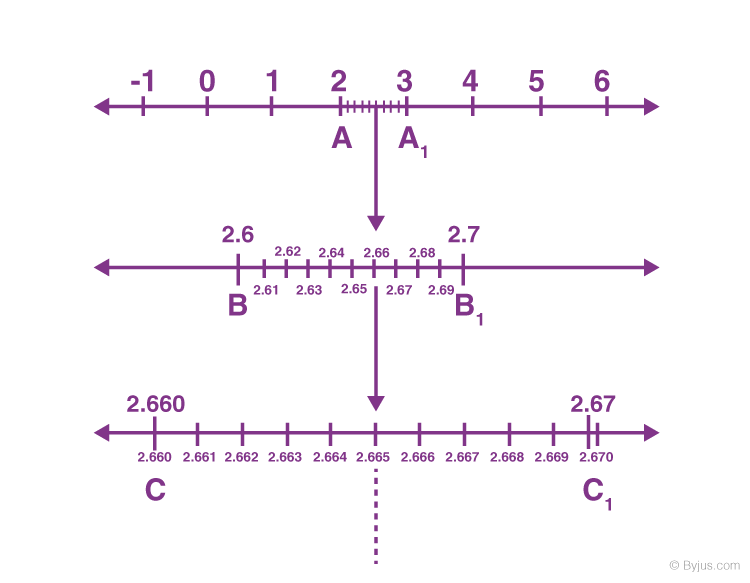Question 2: Visualise the representation of 5.37̅ on the number line upto 5 decimal places, that is upto 5.37777.

Solution:

Clearly 5.37̅ is located between 5 and 6.

Again by successive magnification, and successively decrease 5.37̅ located between 5.3 and 5.4.

For more clarity, divide 5.3 and 5.4 portion of the number line into 10 equal parts and we can see 5.37̅ lies between 5.37 and 5.38.

To visualize 5.37̅ more accurately, divide line segment between 5.37 and 5.38 in ten equal parts.

5.37̅ lies between 5.377 and 5.378.

Again divide above portion between 5.377 and 5.378 into 10 equal parts, which shows 5.37̅ is located closer to 5.3778 than to 5.3777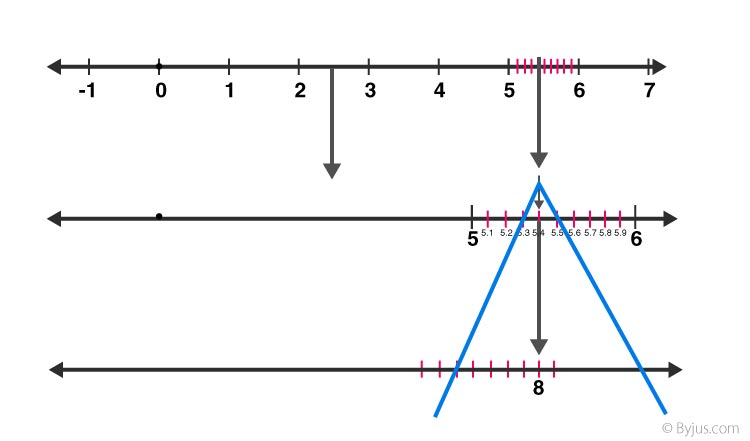### RD Sharma Solutions for Class 9 Maths Chapter 1 Number System

In the 1st chapter of Class 9 RD Sharma Solutions students will study important concepts on Number System as listed below:

• Number System Introduction
• Review of Numbers
• Decimal representation of rational numbers
• Conversion of decimal numbers into rational numbers
• Irrational Numbers
• Some useful results on Irrational Numbers
• Representing Irrational Numbers on the Number Line
• Real Numbers and real number line
• Existence of the square root of a positive real number
• Visualisation of representation of real numbers

### Key benefits of studying RD Sharma Solutions for Class 9 Maths Chapter 1:

• The examples provided in RD Sharma Solutions are given in a step-by-step method for a comfortable and better understanding of concepts.
• The topics provided in the books are explained in a simple and understandable language so that any student can understand the concepts quickly.
• It also provides labelled diagrams and descriptive tables for effective study, which creates interest in learning.
• RD Sharma Solutions strictly follow current CBSE guidelines and provide a lot of questions to solve.

## Frequently Asked Questions on RD Sharma Solutions for Class 9 Maths Chapter 1

### What are the main topics covered in the RD Sharma Solutions for Class 9 Maths Chapter 1?

The main topics covered in the RD Sharma Solutions for Class 9 Maths Chapter 1 are rational and irrational numbers, natural numbers, whole numbers, representation of real numbers and many more. In order to strengthen your basic fundamentals on the number system, students are recommended to follow RD Sharma Solutions thoroughly. Our experts created the solutions effectively in accordance with student’s understanding skills. By practising these solutions regularly, students can score good marks in class tests as well as in Class 9 board exams.

### How many exercises are there in RD Sharma Solutions for Class 9 Maths Chapter 1?

There are six exercises in the RD Sharma Solutions for Class 9 Maths Number system. These solutions of RD Sharma help students in solving the problems adroitly and efficiently. They also focus on cracking the solutions of Maths in such a way that it is easy for the students to understand. The solutions are designed in a simple and descriptive manner by expert faculty so that students can clear their doubts quickly and grasp the concepts with ease.

### Are RD Sharma Solutions for Class 9 Maths Chapter 1 important from an exam point of view?

Yes, the first chapter Number System of RD Sharma Solutions for Class 9 is important from an exam perspective. It contains short answer questions as well as long answer questions to increase the ability of problem-solving. These solutions are created by the BYJU’S expert faculty to help students in the preparation of their examinations. Following these solutions diligently help students to enhance their problem solving and time management skills which are vital for examinations.